# Graphing Spline Predictions in SPSS

I might have around 10 blog posts about using splines in regression models – and you are about to get another. Instead of modeling non-linear effects via polynomial terms (e.g. including x^2, x^3 in a model, etc.), splines are a much better default procedure IMO. For a more detailed mathy exposition on splines and a walkthrough of the functions, see my class notes.

So I had a few questions about applying splines in generalized linear models and including control variables in my prior post (on a macro to estimate the spline terms). These include can you use them in different types of generalized linear models (yes), can you include other covariates into the model (yes). For either of those cases, interpreting the splines are more difficult though. I am going to show an example here of how to do that.

Additionally I have had some recent critiques of my paper on CCTV decay effects. One is that the locations of the knots we chose in that paper is arbitrary. So while that is true, one of the reasons I really like splines is that they are pretty robust – you can mis-specify the knot locations, and if you have enough of them they will tend to fit quite a few non-linear functions. (Also a note on posting pre-prints, despite being rejected twice and under review for around 1.5 years, it has over 2k downloads and a handful of citations. The preprint has more downloads than my typical published papers do.)

So here I am going to illustrate these points using some simulated data according to a particular logistic regression equation. So I know the true effect, and will show how mis-located spline knots still recovers the true effect quite closely. This example is in SPSS, and uses my macro on estimating spline basis.

## Generating Simulated Data

So first in SPSS, I define the location where I am going to save my files. Then I import my Spline macro.

* Example of splines for generalized linear models
* and multiple variables.

DATASET CLOSE ALL.
OUTPUT CLOSE ALL.

* Spline Macro.
FILE HANDLE macroLoc /name = "C:\Users\andre\OneDrive\Desktop\Spline_SPSS_Example".
INSERT FILE = "macroLoc\MACRO_RCS.sps".

Second, I create a set of synthetic data, in which I have a linear changepoint effect at x = 0.42. Then I generate observations according to a particular logistic regression model, with not only the non-linear X effects, but also two covariates Z1 (a binary variable) and Z2 (a continuous variable).

*****************************************************.
* Synthetic data.
SET SEED = 10.
INPUT PROGRAM.
LOOP Id = 1 to 10000.
END CASE.
END LOOP.
END file.
END INPUT PROGRAM.
DATASET NAME Sim.

COMPUTE X = RV.UNIFORM(0,1).
COMPUTE #Change = 0.42.
DO IF X <= #Change.
COMPUTE XDif = 0.
ELSE.
COMPUTE XDif = X - #Change.
END IF.
COMPUTE Z1 = RV.BERNOULLI(0.5).
COMPUTE Z2 = RV.NORMAL(0,1).

DEFINE !INVLOGIT (!POSITIONAL  !ENCLOSE("(",")") )
1/(1 + EXP(-!1))
!ENDDEFINE.

*This is a linear changepoint at 0.42, other variables are additive.
COMPUTE ylogit = 1.1 + -4.3*x + 2.4*xdif + -0.4*Z1 + 0.2*Z2.
COMPUTE yprob = !INVLOGIT(ylogit).
COMPUTE Y = RV.BERNOULLI(yprob).
*These are variables you won't have in practice.
ADD FILES FILE =* /DROP ylogit yprob XDif.
FORMATS Id (F9.0) Y Z1 (F1.0) X Z2 (F3.2).
EXECUTE.
*****************************************************.

## Creating Spline Basis and Estimating a Model

Now like I said, the correct knot location is at x = 0.42. Here I generate a set of regular knots over the x input (which varies from 0 to 1), at not the exact true value for the knot.

!rcs x = X loc = [0.1 0.3 0.5 0.7 0.9].

Now if you look at your dataset, there are 3 new splinex? variables. (For restricted cubic splines, you get # of knots - 2 new variables, so with 5 knots you get 3 new variables here.)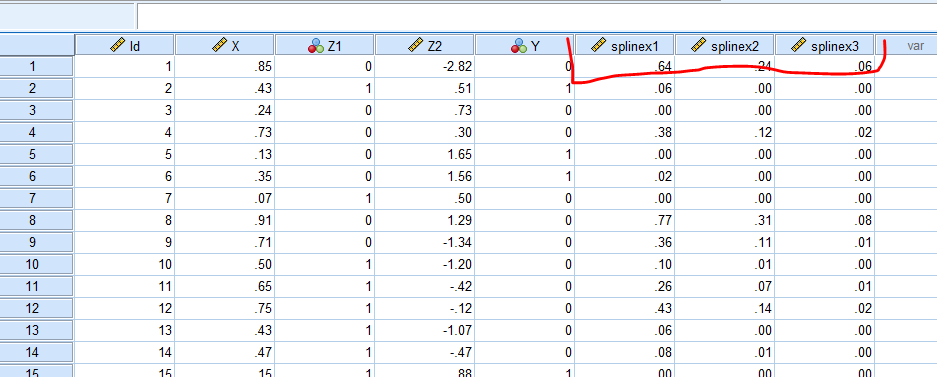We are then going to use those new variables in a logistic regression model. We are also going to save our model results to an xml file. This allows us to use that model to score a different dataset for predictions.

GENLIN Y (REFERENCE=0) WITH X splinex1 splinex2 splinex3 Z1 Z2
/MODEL X splinex1 splinex2 splinex3 Z1 Z2
/OUTFILE MODEL='macroLoc\LogitModel.xml'. 

And if we look at the coefficients, you will see that the coefficients look offhand very close to the true coefficients, minus splinex2 and splinex3. But we will show in a second that those effects should be of no real concern.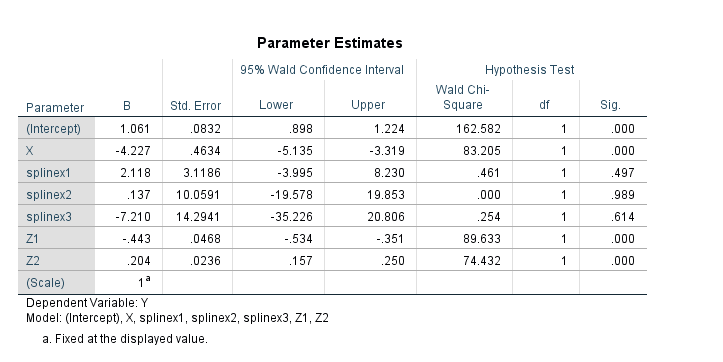## Generating New Data and Plotting Predictions

So you should do this in general with generalized linear models and/or non-linear effects, but to interpret spline effects you can’t really look at the coefficients and know what those mean. You need to make plots to understand what the non-linear effect looks like.

So here in SPSS, I create a new dataset, that has a set of regularly sampled locations along X, and then set the covariates Z1=1 and Z2=0. These set values you may choose to be at some average, such as mean, median, or mode depending on the type of covariate. So here since Z1 can only take on values of 0 and 1, it probably doesn’t make sense to choose 0.5 as the set value. Then I recreate my spline basis functions using the exact sample macro call I did earlier.

INPUT PROGRAM.
LOOP #xloc = 0 TO 300.
COMPUTE X = #xloc/300.
END CASE.
END LOOP.
END FILE.
END INPUT PROGRAM.
DATASET NAME Fixed.
COMPUTE Z1 = 1.
COMPUTE Z2 = 0.
EXECUTE.
DATASET ACTIVATE Fixed.

*Redoing spline variables.
!rcs x = X loc = [0.1 0.3 0.5 0.7 0.9].

Now in SPSS, we score this dataset using our prior model xml file we saved. Here this generates the predicted probability from our logistic model.

MODEL HANDLE NAME=LogitModel FILE='macroLoc\LogitModel.xml'.
COMPUTE PredPr = APPLYMODEL(LogitModel, 'PROBABILITY', 1).
EXECUTE.
MODEL CLOSE NAME=LogitModel.

And to illustrate how close our model is, I generate what the true predicted probability should be based on our simulated data.

*Lets also do a line for the true effect to show how well it fits.
COMPUTE #change = 0.42.
DO IF X <= #change.
COMPUTE xdif = 0.
ELSE.
COMPUTE xdif = (X - #change).
END IF.
EXECUTE.
COMPUTE ylogit = 1.1 + -4.3*x + 2.4*xdif + -0.4*Z1 + 0.2*Z2.
COMPUTE TruePr = !INVLOGIT(ylogit).
FORMATS TruePr PredPr X (F2.1).
EXECUTE.

And now we can put these all into one graph.

DATASET ACTIVATE Fixed.
GGRAPH
/GRAPHDATASET NAME="graphdataset" VARIABLES=X PredPr TruePr
/FRAME INNER=YES
/GRAPHSPEC SOURCE=INLINE.
BEGIN GPL
SOURCE: s=userSource(id("graphdataset"))
DATA: X=col(source(s), name("X"))
DATA: PredPr=col(source(s), name("PredPr"))
DATA: TruePr=col(source(s), name("TruePr"))
GUIDE: axis(dim(1), label("X"))
GUIDE: axis(dim(2), label("Prob"))
SCALE: cat(aesthetic(aesthetic.shape), map(("PredPr",shape.solid),("TruePr",shape.dash)))
ELEMENT: line(position(X*PredPr), shape("PredPr"))
ELEMENT: line(position(X*TruePr), shape("TruePr"))
END GPL.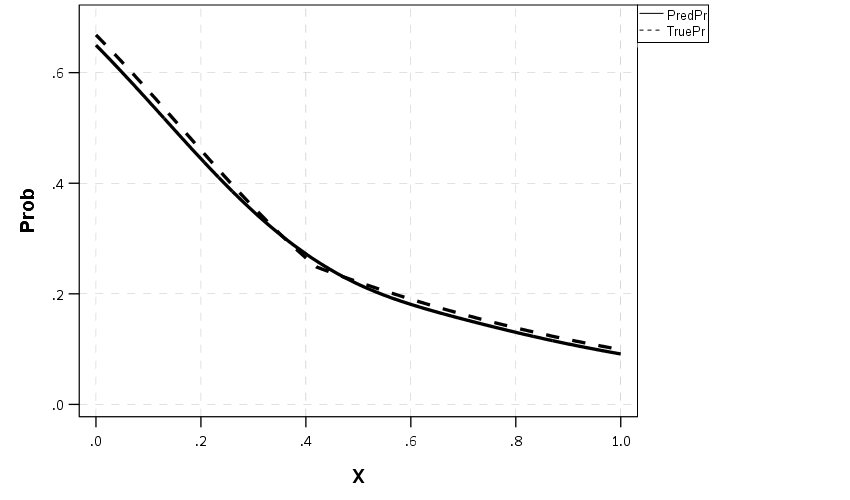So you can see that even though I did not choose the correct knot location, my predictions are nearly spot on with what the true probability should be.

## Generating Predictions Over Varying Inputs

So in practice you can do more complicated models with these splines, such as allowing them to vary over different categories (e.g. interactions with other covariates). Or you may simply want to generate predicted plots such as above, but have a varying set of inputs. Here is an example of doing that; for Z1 we only have two options, but for Z2, since it is a continuous covariate we sample it at values of -2, -1, 0, 1, 2, and generate lines for each of those predictions.

*****************************************************.
* Can do the same thing, but vary Z1/Z2.

DATASET ACTIVATE Sim.
DATASET CLOSE Fixed.

INPUT PROGRAM.
LOOP #xloc = 0 TO 300.
LOOP #z1 = 0 TO 1.
LOOP #z2 = -2 TO 2.
COMPUTE X = #xloc/300.
COMPUTE Z1 = #z1.
COMPUTE Z2 = #z2.
END CASE.
END LOOP.
END LOOP.
END LOOP.
END FILE.
END INPUT PROGRAM.
DATASET NAME Fixed.
EXECUTE.
DATASET ACTIVATE Fixed.

*Redoing spline variables.
!rcs x = X loc = [0.1 0.3 0.5 0.7 0.9].

MODEL HANDLE NAME=LogitModel FILE='macroLoc\LogitModel.xml'.
COMPUTE PredPr = APPLYMODEL(LogitModel, 'PROBABILITY', 1).
EXECUTE.
MODEL CLOSE NAME=LogitModel.

FORMATS Z1 Z2 (F2.0) PredPr X (F2.1).
VALUE LABELS Z1
0 'Z1 = 0'
1 'Z1 = 1'.
EXECUTE.

*Now creating a graph of the predicted probabilities over various combos.
*Of input variables.
DATASET ACTIVATE Fixed.
GGRAPH
/GRAPHDATASET NAME="graphdataset" VARIABLES=X PredPr Z1 Z2
/FRAME INNER=YES
/GRAPHSPEC SOURCE=INLINE.
BEGIN GPL
SOURCE: s=userSource(id("graphdataset"))
DATA: X=col(source(s), name("X"))
DATA: PredPr=col(source(s), name("PredPr"))
DATA: TruePr=col(source(s), name("TruePr"))
DATA: Z1=col(source(s), name("Z1"), unit.category())
DATA: Z2=col(source(s), name("Z2"), unit.category())
COORD: rect(dim(1,2), wrap())
GUIDE: axis(dim(1), label("X"))
GUIDE: axis(dim(2), label("Predicted Probability"))
GUIDE: axis(dim(3), opposite())
GUIDE: legend(aesthetic(aesthetic.color), label("Z2"))
SCALE: cat(aesthetic(aesthetic.color), map(("-2",color."8c510a"),("-1",color."d8b365"),
("0",color."f6e8c3"), ("1",color."80cdc1"), ("2",color."018571")))
ELEMENT: line(position(X*PredPr*Z1), color(Z2))
END GPL.
*****************************************************.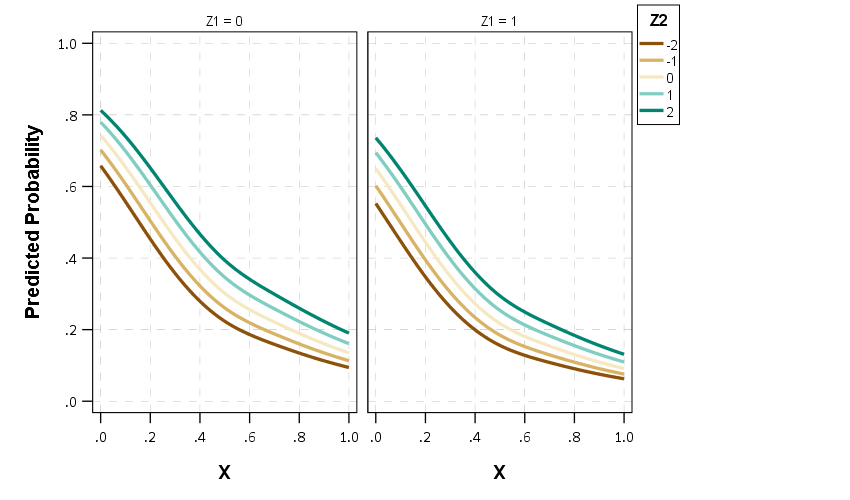So between all of these covariates, the form of the line does not change much (as intended, I simulated the data according to an additive model).

If you are interested in drawing more lines for Z2, you may want to use a continuous color scale instead of a categorical one (see here for a similar example).

# Outliers in Distributions

If you google ‘outlier’, all of the results that come up are in terms of individual observation outliers. So if you have a set of transaction data that is 10, 20, 30, 8000, the singlet observation 8000 is an outlier. But for many situations with transaction data, you don’t want to examine individual outlier incidents, but look for systematic patterns. For example, if I am looking at healthcare insurance claims for my work, a single claim that is $100,000 is actually not that rare. But if we have a hospital that has mostly$100,000 claims for a specific treatment, whereas another with similar cases has a range of $50,000 to$100,000, that may signal there is some funny business going on.

There is no singular way to examine outliers in distribution. A plain old t-test of mean differences may make sense for some situations. But a generally more useful way IMO to think about the problem is to examine the distribution of the outcome in CDF space, as opposed to looking at particular moments of the distribution. A t-test basically only looks at the differences in means for the distributions, whereas examining the CDF we are looking for weird patterns at any point in the distribution.

Here is an example of comparing the cost of hospital stays (per length of stay), for a hospital compared to all others from the same datasource (details on the data in a sec). The way to read this graph is that at 10^3 (so $1000 per day claims) for facility 1458, we have around 20% of the claims data are below this value. For the rest of the hospital data, a larger proportion of claims are under a thousand dollars, more like 25%. Since the red line is always below the black line, it also means that the claims at this hospital are pretty much always larger than the claims at all the other hospitals.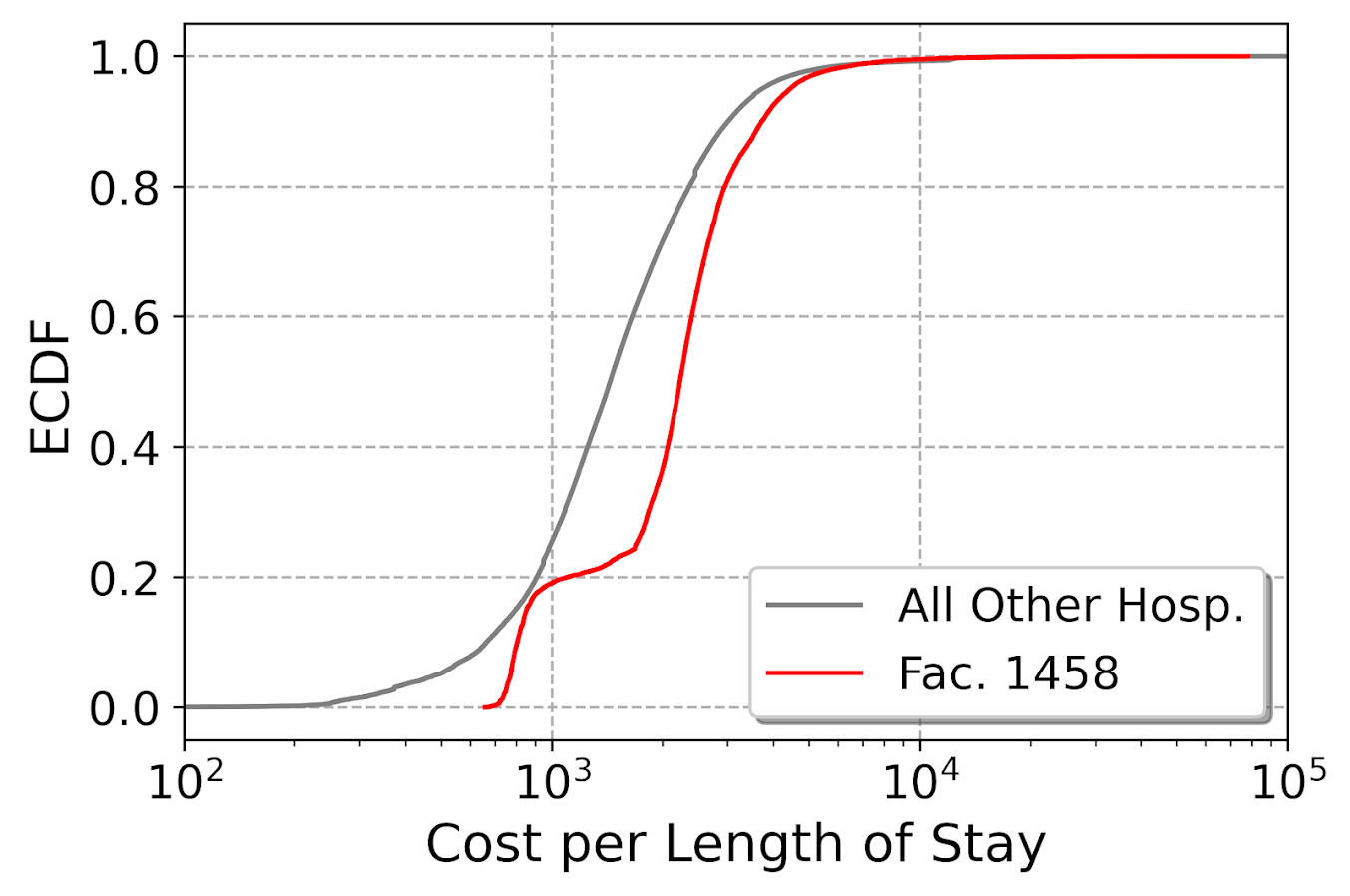For this example analysis, I am using data from New York State health insurance claims data (SPARC). I have posted python code to replicate here (note if you cannot access dropbox links, feel free to email and I will forward). Here I am specifically analyzing medical, in-patient insurance claims (I dropped surgical claims) for around 300+ hospitals. There are quite a few claims in this data, over 2 million, and the majority of hospitals have plenty of claims to examine (so no hospitals with only 10 claims). I also specifically examine costs per length of stay. Initially I just examined costs, but will get to why I changed to the normalized version towards the end of the post. # Analysis of CDF Outliers So first what I did was attempt to do a leave-one-out type stat test using the Kolmogorov-Smirnov test. This is a test that looks at the maximum vertical difference between the CDFs I showed earlier. I should have known better though. Given this large of sample size, even with multiple comparison adjustments for false discovery rate, every hospital was considered an outlier. This is sort of the curse of null hypothesis significance testing, it is either underpowered, so you get null results when things should really be flagged, or with large samples everything is flagged. So what I did first was make a graph of all the different CDFs for each individual hospital. You can see from this plot we have a mass of the distribution that looks very similar in shape, but is shifted left or right. (Hospitals can bill different values, i.e. casemix, so can have the same types of events but have different bills, so that is normal.) But then we have a few outliers really stick out.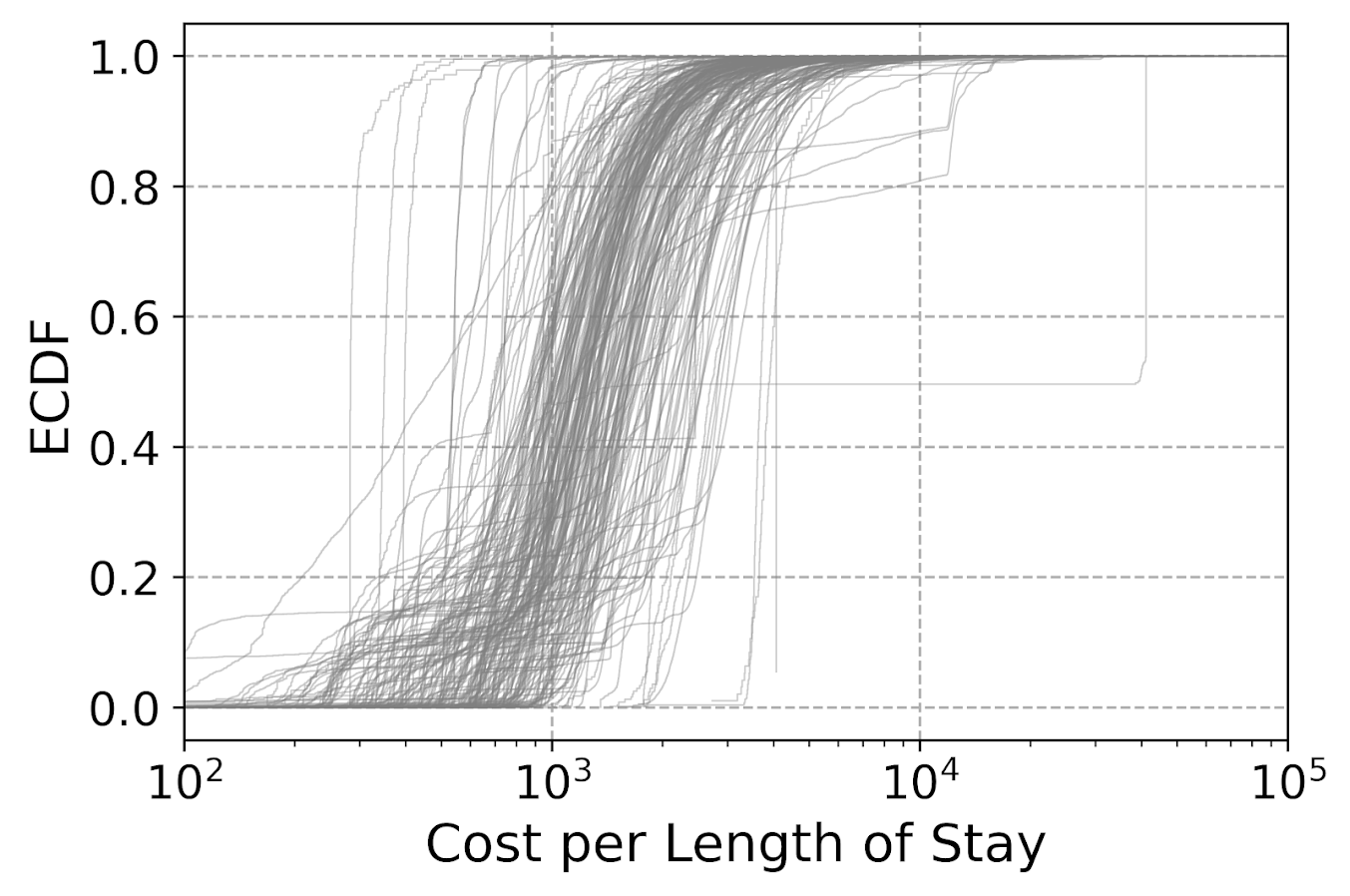To characterize the central mass in this image, what I did was calculate each empirical CDF for each hospital (over 300 in this sample). Then I estimated the CDF for each hospital at a sample of points logspace distributed between$100 to $100,000. Then I took the 90% distribution between the ECDF values. This is easier to show than to say, so in the below pic the grey area is the 90% region for the CDFs. Then you can do stats to see how hospitals may fall outside that band.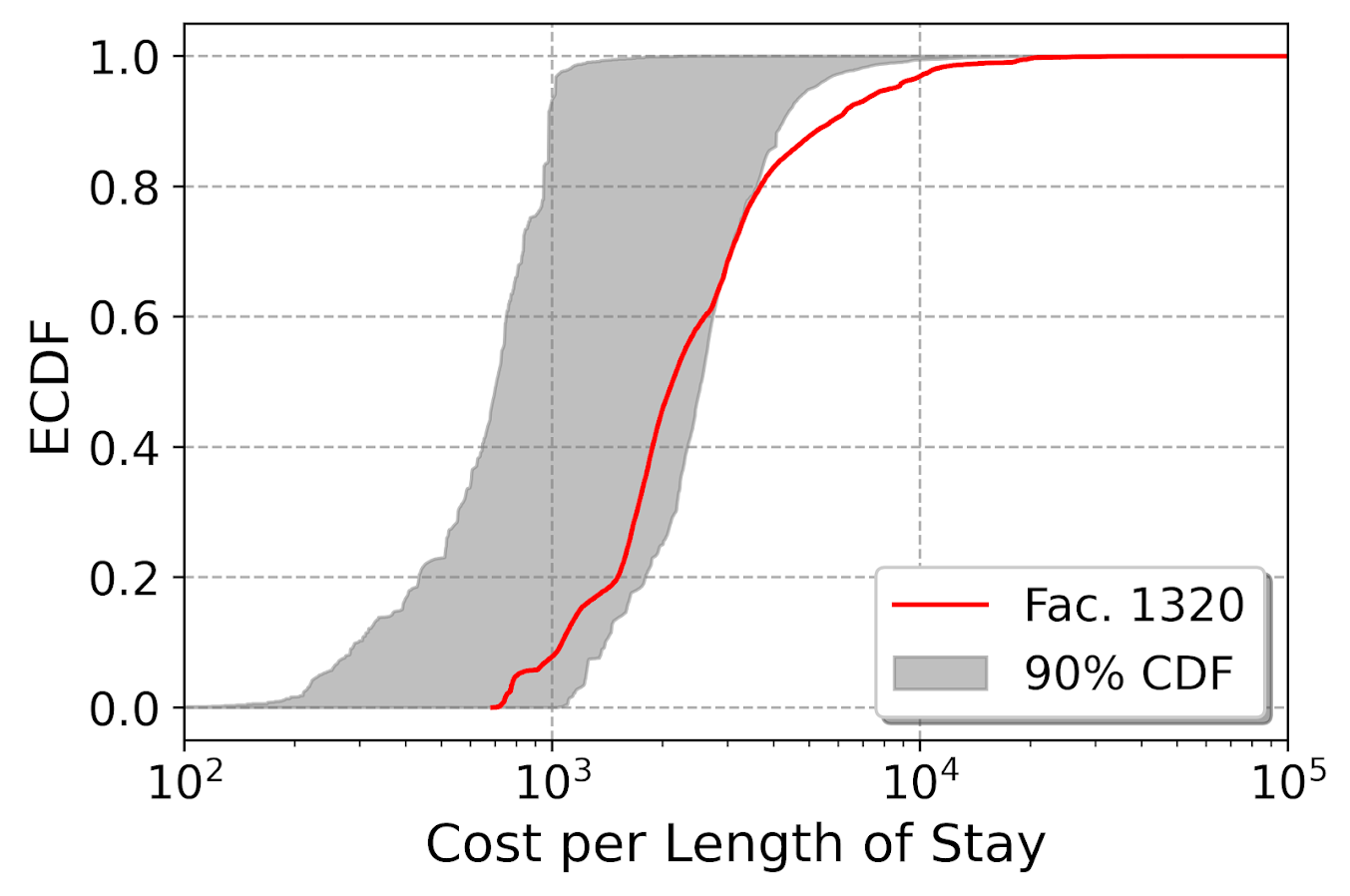So here 1320 is looking good until around 60% of the distribution, and then it is shifted right. There is a kink in the CDF as well, so this suggests really a set of different types of claims, and in that second group it is the outlier. 1320 was the hospital that had the most sample points outside of my grey coverage area, but you could also do outliers in terms of the distance between those two lines (again like a KS test stat), or in the area between those two lines (that is like a version of the Wasserstein distance only considering above/below moves). So here is the hospital that has the largest distance below the band (above the band signals that a hospital has lower claims on average):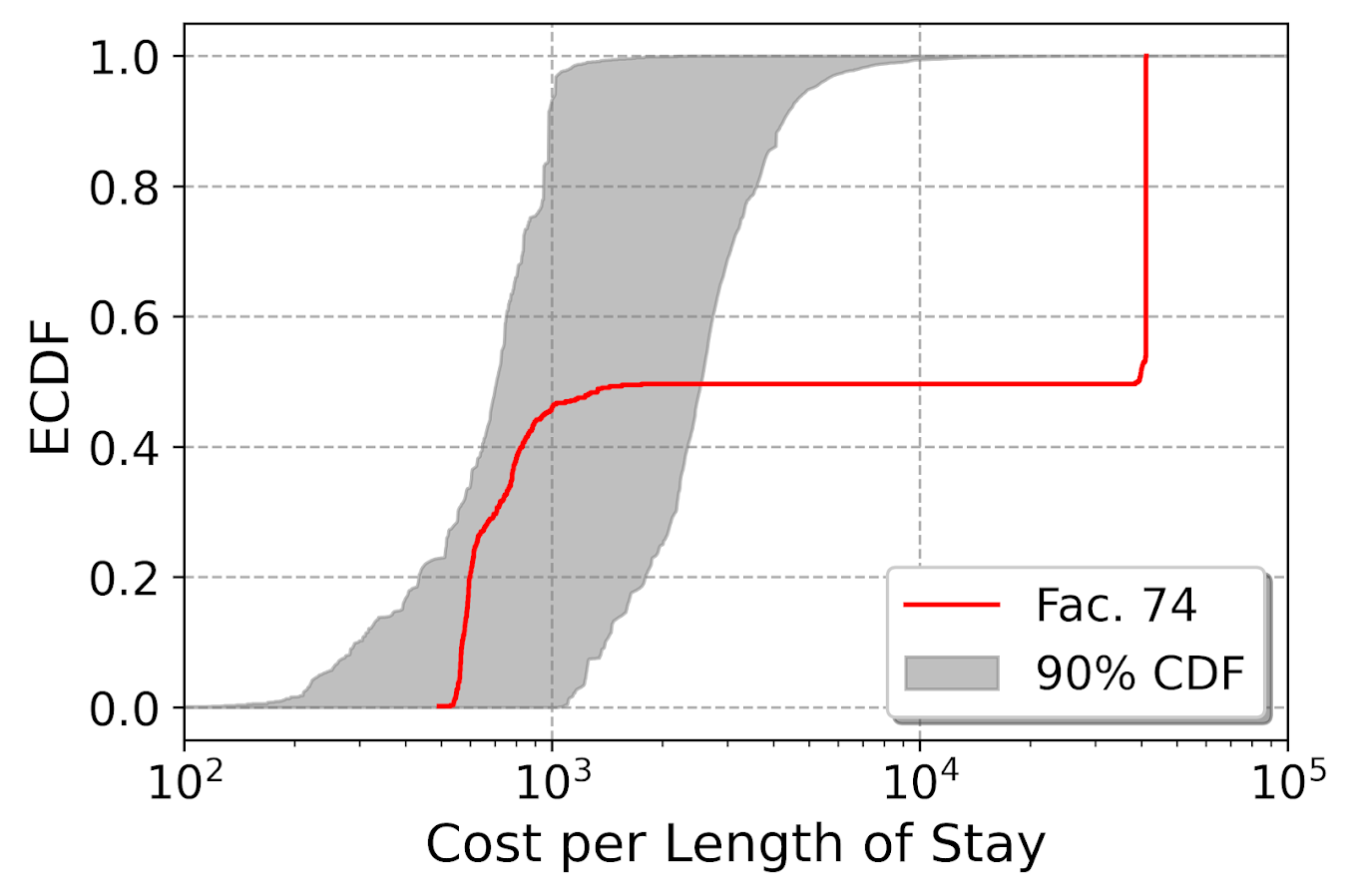Flat lines horizontally signal an absence of data, whereas vertical lines signal a set of claims with the exact same bill. So here we have a set of claims around$1000 per day that look normal, then an abnormal absence of data from $1,000 to$10,000. Then a large spike of claims that end up being around 45k per day. So this is looking at the distribution relative to other hospitals, but a few examples I am familiar with look for these flat/vertical spikes in the CDF to identify fraud. Mike Maltz has an example of identifying collusion in bids. In another, Chris Stucchio identifies spikes in transaction data signaling potential fraud. Here I am just doing a test relative to other data to identify weird curves, not just flat lines though. One limitation of this analysis I have conducted here is that it does not take into account the nature of the claims data. So say you had a hospital that specializes in cancer treatment, it may be totally normal for them to have claims that are higher value overall than a more typical hospital that spreads claims among a wider variety of types of visits/treatments. Initially I analyzed just the cost data, and it identified a few big outliers that ended up being hospice/nursing homes. So they had really high dollar value claims, but also really long stays. So when analyzing the claim per length of say, they were totally normal in that central mass. So ultimately there could be other characteristics in the types of claims hospitals submit that could explain the weird CDF. One way to take that into account is to do a conditional model for the claims, and then do the ECDF tests on those conditional models. One way may be to look at the residuals for each individual hospital, another would be to draw a matched comparison sample. (Greg Ridgeway did this when examining police behavior in the NYPD.) That would be like making a single comparison line (like my initial black/red line graph). So controlling the false discovery after that will be tough with larger samples (again the typical KS test, even with a matched sample, will likely always reject). So wondering if there is another machine learning way to identify outliers in CDF space, like a mashup of isolation forests and conditional density forests. Essentially I want to fit a model to draw those grey CDF bands, instead of relying on my sample of hospitals to draw the grey band in those latter plots. # Mapping attitudes paper published My paper (joint work with Jasmine Silver, Rob Worden, and Sarah McLean), Mapping attitudes towards the police at micro places, has been published in the most recent issue of the Journal of Quantitative Criminology. Here is the abstract: Objectives: We examine satisfaction with the police at micro places using data from citizen surveys conducted in 2001, 2009 and 2014 in one city. We illustrate the utility of this approach by comparing micro- and meso-level aggregations of policing attitudes, as well as by predicting views about the police from crime data at micro places. Methods: In each survey, respondents provided the nearest intersection to their address. Using that geocoded survey data, we use inverse distance weighting to map a smooth surface of satisfaction with police over the entire city and compare the micro-level pattern of policing attitudes to survey data aggregated to the census tract. We also use spatial and multi-level regression models to estimate the effect of local violent crimes on attitudes towards police, controlling for other individual and neighborhood level characteristics. Results: We demonstrate that there are no systematic biases for respondents refusing to answer the nearest intersection question. We show that hot spots of dissatisfaction with police do not conform to census tract boundaries, but rather align closely with hot spots of crime. Models predicting satisfaction with police show that local counts of violent crime are a strong predictor of attitudes towards police, even above individual level predictors of race and age. Conclusions: Asking survey respondents to provide the nearest intersection to where they live is a simple approach to mapping attitudes towards police at micro places. This approach provides advantages beyond those of using traditional neighborhood boundaries. Specifically, it provides more precise locations police may target interventions, as well as illuminates an important predictor (i.e., nearby violent crimes) of policing attitudes. And this was one of my favorites to make maps. We show how to take surveys and create analogs of hot spot maps of negative sentiment towards police. We do this via asking individuals to list their closest intersection (to still give some anonymity), and then create inverse distance weighted maps of negative attitudes towards police.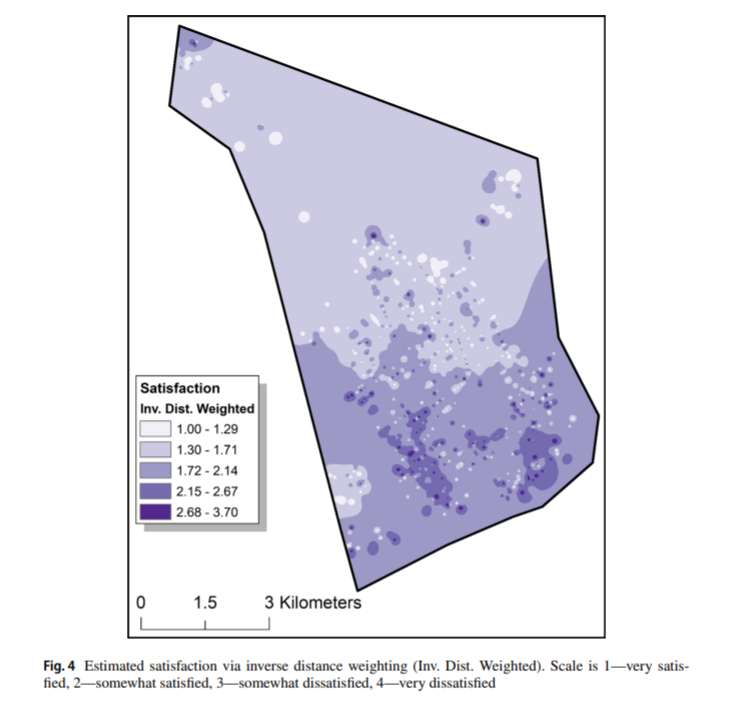We also find in this work that nearby crimes are the biggest factor in predicting negative sentiment towards police. This hints that past results aggregating attitudes to neighborhoods is inappropriate, and that police reducing crime is likely to have the best margin in terms of making people more happy with the police in general.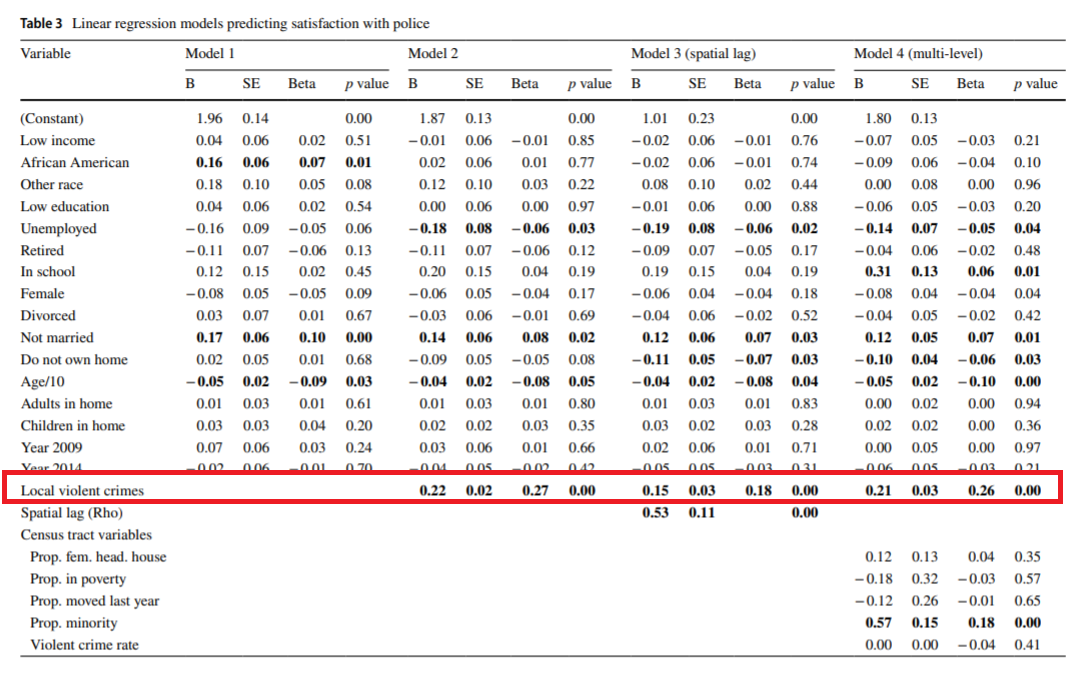As always, feel free to reach out for a copy of the paper if you cannot access JQC. (Or you could go a view the pre-print.) # Overview of DataViz books Keith McCormick the other day on LinkedIn the other day made a post/poll on his favorite data viz books. (I know Keith because I contributed a chapter on geospatial data analysis in SPSS in Keith and Jesus Salcedo’s book, SPSS Statistics for Data Analysis and Visualization, and Jon Peck contributed a chapter as well.) One thing about this topical area is that there isn’t a standard Data Viz 101 curriculum. So if you pick up Statistics 101 books, they will cover pretty much all the same material (normal distribution, central limit theorem, t-tests, regression). It isn’t 100% overlap (some may spend more time on elementary probability, and others may cover ANOVA), but for someone learning the material there isn’t much point in reading multiple introductory stats books. This is not so with the Data Viz books in Keith’s picture – they are very different in content. As I have read quite a few different books on the topic over the years I figured I would give my breakdown of the various books. # Albert Cairo’s The Functional Art While my list is not in rank order, I am putting Cairo’s book first for a reason. Although there is not a Data Viz 101 curriculum, this book is the closest thing to it. Cairo goes through in short order various cognitive aspects on how we view the world that are fundamental to building good data visualizations. This includes things like it is easier to compare lengths along a common axis, and that we can perceive rank order to color saturation, but not to a color’s hue. It is also enjoyable to read because of all the great journalistic examples. I did not care so much for the interviews at the back, and I don’t like the cover. But if I did a data viz course for undergrads in social sciences (Cairo developed this for journalism students), I would likely assign this book. But despite being very accessible, he covers a broad spectrum of both simple graphs and complicated scientific diagrams. For this review many of these authors have other books. So I haven’t read Cairo’s The Truthful Art, so I cannot comment on it. # Edward Tufte’s The Visual Display of Quantitative Information Tufte’s book was the first data viz book I bought in grad school. I initially invested in it as he had a chapter on a critique of powerpoint presentations, which is very straightforward and provides practical advice on what not to do. Most of the critiques of this book are that it is mostly just a collection of Tufte’s opinions about creating minimalist, dense, scientific graphs. So while Cairo dives into the science of perception, Tufte is just riffing his opinions. His opinions are based on his experience though, and they are good! I believe I have read all of Tufte’s other books as well, but this is the only one that made much of an impression on me (some of his others go beyond graphs, and talk about UI design). I gobbled it up in only two days when I first started reading it, and so if I were stuck on an island with one book scenario I would choose this one over the others I list here (although again think Cairo’s book is the best to start with for most folks). So for scientists I think it is a good investment and an enjoyable read overall. # Nathan Yau’s Visualize This Of all the books I review, Yau’s is the only how-to actually make graphs in software. Unfortunately, much of Yau’s programmatic advice was outdated already when it was published (e.g. flash was already going by the wayside). So while he has many great examples of creating complicated and beautiful data visualizations, the process he outlines to make them are overly complicated IMO (such as using python to edit parts of a pre-made SVG map). It is a good book for examples no doubt, and maybe you can pick up a few tricks in terms of post editing charts in a vector graphics program, such as Illustrator or Inkscape (most examples are making graphs in base R and then exporting to edit finishing touches). In terms of making a how-to book it is really hard. Yau I am sure has updates on his Flowing Data website to make charts (and maybe his newer book is better). But I don’t think I would recommend investing in this book for anything beyond looking at pretty examples of data viz. # Stephen Kosslyn’s Graph Design for the Eye and Mind The prior books all contained complicated, dense, scientific graphs. Kosslyn’s book is specifically oriented to making corporate slide decks/powerpoints, in which the audience is not academic. But his advice is mostly backed on his understanding of the psychology (he relegates extensive endnotes to point to scientific lit, to avoid cluttering up the basic book). He has as few gems of advice I admit, such as it isn’t the number of lines in a graph that make it complicated, but really the number of unique profiles. But then he has some pieces I find bizarre, such as saying pie charts are OK because they are so popular (so have survived a Darwinian survival process in terms of being presented to business people). I would stick with Tufte’s powerpoint advice (and later will mention a few other books related to giving presentations), as opposed to recommending this book. # Alan MacEachren How maps work: Representation, visualization, and design MacEachren’s book is encyclopedic in terms of scientific literature on design aspects of both cartography, as well as the psychological literature. So it is like reading an encyclopedia (not 100% sure if I ever finished it front to back to be honest). I would start here if you are interested in designing cognitive experiments to test certain graphs/maps. I think MacEachren pooling from cartography and psychology ends up being a better place to start than say Colin Ware’s Information Visualization (but it is close). They are both very academically oriented though. # Leland Wilkinson’s The Grammar of Graphics I used SPSS for along time when I read this book, so I was already quite familiar with the grammar of graphics in terms of creating graphs in SPSS. That pre-knowledge helped me digest Wilkinson’s material I believe. Nick Cox has a review of this book, and for this one he notes that the audience for this book is hard to pin down. I agree, in that you need to be pretty far along already in terms of making graphs to be able to really understand the material, and as such it is not clear what the benefit is. Even for power users of SPSS, much of the things Wilkinson talks about are not implemented in SPSS’s GGRAPH language, so they are mostly just on paper. (Note Nick has a ton of great reviews on Amazon as well for various data viz books. He is a good place to start to decide if you want to purchase a book. For example the worst copy-edited book I have ever seen is Andy Kirk’s via Packt publishing, and Nick notes how poorly it is copy-edited in his review.) Here is an analogy I think is apt for Wilkinson’s book – if we are talking about cars, you may have a book on the engineering of the car, and another on how to actually drive the car. Knowing how pistons work in a combustible engine does not help you drive a car, but helps you build one. Wilkinson’s book is more about the engineering of a graph from an algebraic perspective. At the fringes it helps in thinking about the components of graphs, but doesn’t really give any advice about what graph to make in-and-of itself, nor what is a good graph or a bad graph. Note that the R library ggplot2, is actually quite a bit different than Leland’s vision. It is simpler, in that Wickham essentially drops the graph algebra part, so you specify the axes directly, whereas in Wilkinson’s you just say X*Y*Z, and depending on other aspects of the grammer this may produce a 3d scatterplot, a facet gridded scatterplot, a clustered bar chart, etc. I think Wickham was right to make that design choice, but in doing so it really isn’t an implementation of what Wilkinson was talking about in this book. # Jacques Bertin’s Semiology of Graphics: Diagrams, Networks, Maps Bertin’s book is an attempt to make a dictionary of terms for different aspects of graphs. So it is a bit in the weeds. One unique aspect of Bertin is that he discusses titles and labels for graphs, although I wouldn’t go as far as saying that his discussion leads to straightforward advice. I find Wilkinson’s grammer of graphics a more useful way to overall think about the components of a graph, although Bertin is more encyclopedic in coverage of different types of graphs and maps in the wild. # Short notes on various other books Most of these books (with the exception of Nathan Yau’s) are not how-to actually write code to make graphs. For those that use R, there are two good options though. Hadley Wickham’s ggplot2: Elegant Graphics for Data Analysis (Use R!) was really good at the time (I am not sure if the newer version is more up to date though, like any software it changes over time so the older one I know is out of date for many different code examples). And though I’ve only skimmed it, Kieran Healy’s Data Visualization: A practical introduction is free and online and looks good (and also for those interested in criminal justice examples Jacob Kaplan has examples in R as well, Crime by the Numbers). So those later two I know are good in terms of being up to date. For python I just suggest using google (Jake VanderPlas has a book that looks good, and his website is really good). For excel I really like Jorge Camões work (his book is Data at Work, which I don’t think I’ve read, but have followed his website for along time). In terms of scientific presentations (which covers both graphs and text), I’ve highly suggested in the past Trees, maps, and theorems. This is similar in spirit to Tufte’s minimalist style, but gives practical advice on slides, writing, and presentations. Jon Schwabish’s book, Better Presentations: A Guide for Scholars, Researchers, and Wonks, is very good as well in terms of direct advice. I think for folks in academia I would say go for Doumont’s book, and for those in corporate environment go for Schwabish’s. Stephen Few’s books deserve a mention here as well, such as Show me the numbers. Stephen is the only one to do a deep dive into the concept of dashboards. Stephen’s advice is very straightforward and more oriented towards a corporate type environment, not so much a scientific one (although it isn’t bad advice for scientists, ditto for Schwabish, just stating more so for an understanding of the intended audience). I could go on forever, Tukey’s EDA, Calvin Schmid’s book on how to draw graphs with actual splines! How to lie with statistics and how to lie with maps. So many to choose from. But I think if you are starting out in a data oriented role in which you need to make graphs, I would suggest starting with Cairo’s book, then get Tufte to really get some artistic motivation and a good review of bad powerpoint practices. The rest are more advanced material for study though. # Making smoothed scatterplots in python The other day I made a blog post on my notes on making scatterplots in matplotlib. One big chunk of why you want to make scatterplots though is if you are interested in a predictive relationship. Typically you want to look at the conditional value of the Y variable based on the X variable. Here are some example exploratory data analysis plots to accomplish that task in python. I have posted the code to follow along on github here, in particular smooth.py has the functions of interest, and below I have various examples (that are saved in the Examples_Conditional.py file). # Data Prep First to get started, I am importing my libraries and loading up some of the data from my dissertation on crime in DC at street units. My functions are in the smooth set of code. Also I change the default matplotlib theme using smooth.change_theme(). Only difference from my prior posts is I don’t have gridlines by default here (they can be a bit busy). ################################# import pandas as pd import numpy as np import matplotlib.pyplot as plt import seaborn as sns import statsmodels.api as sm import os import sys mydir = r'D:\Dropbox\Dropbox\PublicCode_Git\Blog_Code\Python\Smooth' data_loc = r'https://dl.dropbox.com/s/79ma3ldoup1bkw6/DC_CrimeData.csv?dl=0' os.chdir(mydir) #My functions sys.path.append(mydir) import smooth smooth.change_theme() #Dissertation dataset, can read from dropbox DC_crime = pd.read_csv(data_loc) ################################# # Binned Conditional Plots The first set of examples, I bin the data and estimate the conditional means and standard deviations. So here in this example I estimate E[Y | X = 0], E[Y | X = 1], etc, where Y is the total number of part 1 crimes and x is the total number of alcohol licenses on the street unit (e.g. bars, liquor stores, or conv. stores that sell beer). The function name is mean_spike, and you pass in at a minimum the dataframe, x variable, and y variable. I by default plot the spikes as +/- 2 standard deviations, but you can set it via the mult argument. #################### #Example binning and making mean/std dev spike plots smooth.mean_spike(DC_crime,'TotalLic','TotalCrime') mean_lic = smooth.mean_spike(DC_crime,'TotalLic','TotalCrime', plot=False,ret_data=True) ####################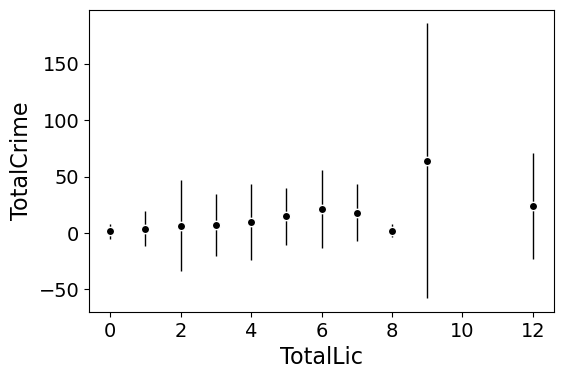This example works out because licenses are just whole numbers, so it can be binned. You can pass in any X variable that can be binned in the end. So you could pass in a string for the X variable. If you don’t like the resulting format of the plot though, you can just pass plot=False,ret_data=True for arguments, and you get the aggregated data that I use to build the plots in the end. mean_lic = smooth.mean_spike(DC_crime,'TotalLic','TotalCrime', plot=False,ret_data=True) Another example I am frequently interested in is proportions and confidence intervals. Here it uses exact binomial confidence intervals at the 99% confidence level. Here I clip the burglary data to 0/1 values and then estimate proportions. #################### #Example with proportion confidence interval spike plots DC_crime['BurgClip'] = DC_crime['OffN3'].clip(0,1) smooth.prop_spike(DC_crime,'TotalLic','BurgClip') ####################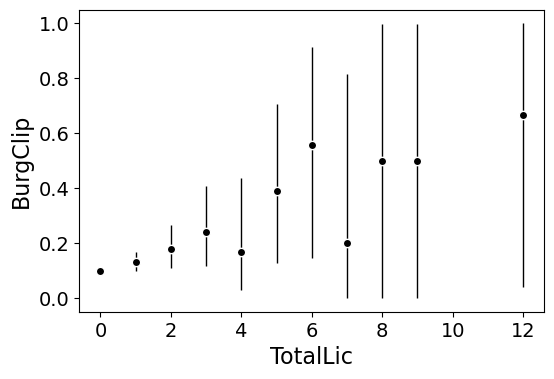A few things to note about this is I clip out bins with only 1 observation in them for both of these plots. I also do not have an argument to save the plot. This is because I typically only use these for exploratory data analysis, it is pretty rare I use these plots in a final presentation or paper. I will need to update these in the future to jitter the data slightly to be able to superimpose the original data observations. The next plots are a bit easier to show that though. # Restricted Cubic Spline Plots Binning like I did prior works out well when you have only a few bins of data. If you have continuous inputs though it is tougher. In that case, typically what I want to do is estimate a functional relationship in a regression equation, e.g. Y ~ f(x), where f(x) is pretty flexible to identify potential non-linear relationships. Many analysts are taught the loess linear smoother for this. But I do not like loess very much, it is often both locally too wiggly and globally too smooth in my experience, and the weighting function has no really good default. Another popular choice is to use generalized additive model smoothers. My experience with these (in R) is better than loess, but they IMO tend to be too aggressive, and identify overly complicated functions by default. My favorite approach to this is actually then from Frank Harrell’s regression modeling strategies. Just pick a regular set of restricted cubic splines along your data. It is arbitrary where to set the knot locations for the splines, but my experience is they are very robust (so chaning the knot locations only tends to change the estimated function form by a tiny bit). I have class notes on restricted cubic splines I think are a nice introduction. First, I am going to make the same dataset from my class notes, the US violent crime rate from 85 through 2010. years = pd.Series(list(range(26))) vcr = [1881.3, 1995.2, 2036.1, 2217.6, 2299.9, 2383.6, 2318.2, 2163.7, 2089.8, 1860.9, 1557.8, 1344.2, 1268.4, 1167.4, 1062.6, 945.2, 927.5, 789.6, 734.1, 687.4, 673.1, 637.9, 613.8, 580.3, 551.8, 593.1] yr_df = pd.DataFrame(zip(years,years+1985,vcr), columns=['y1','years','vcr']) I have a function that allows you to append the spline basis to a dataframe. If you don’t pass in a data argument, in returns a dataframe of the basis functions. #Can append rcs basis to dataframe kn = [3.0,7.0,12.0,21.0] smooth.rcs(years,knots=kn,stub='S',data=yr_df)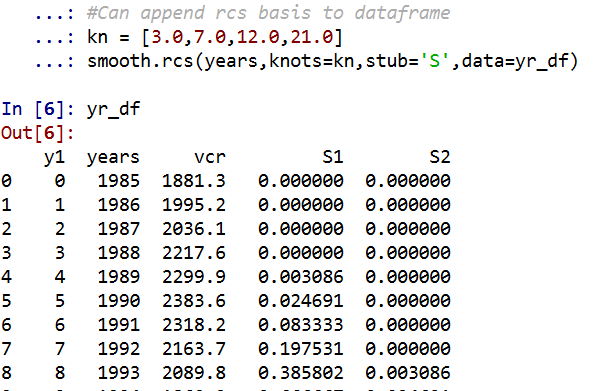I also have in the code set Harrell’s suggested knot locations for the data. This ranges from 3 to 7 knots (it will through an error if you pass a number not in that range). This here suggests the locations [1.25, 8.75, 16.25, 23.75]. #If you want to use Harrell's rules to suggest knot locations smooth.sug_knots(years,4) Note if you have integer data here these rules don’t work out so well (can have redundant suggested knot locations). So Harell’s defaults don’t work with my alcohol license data. But it is one of the reasons I like these though, I just pick regular locations along the X data and they tend to work well. So here is a regression plot passing in those knot locations kn = [3.0,7.0,12.0,21.0] I defined a few paragraphs ago, and the plot does a few vertical guides to show the knot locations. #RCS plot smooth.plot_rcs(yr_df,'y1','vcr',knots=kn)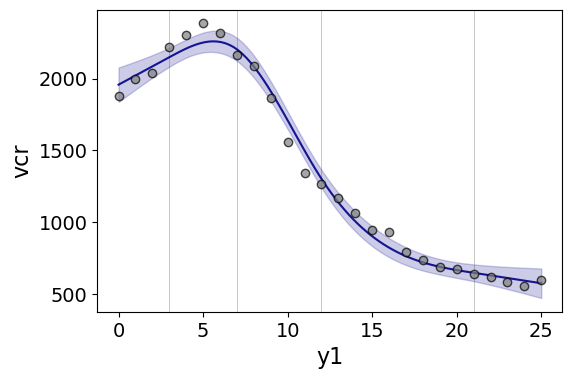Note that the error bands in the plot are confidence intervals around the mean, not prediction intervals. One of the nice things though about this under the hood, I used statsmodels glm interface, so if you want you can change the underlying link function to Poisson (I am going back to my DC crime data here), you just pass it in the fam argument: #Can pass in a family argument for logit/Poisson models smooth.plot_rcs(DC_crime,'TotalLic','TotalCrime', knots=[3,7,10,15], fam=sm.families.Poisson(), marker_size=12)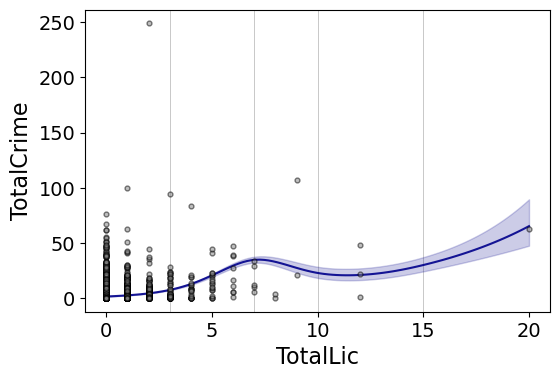This is a really great example for the utility of splines. I will show later, but a linear Poisson model for the alcohol license effect extrapolates very poorly and ends up being explosive. Here though the larger values the conditional effect fits right into the observed data. (And I swear I did not fiddle with the knot locations, there are just what I picked out offhand to spread them out on the X axis.) And if you want to do a logistic regression: smooth.plot_rcs(DC_crime,'TotalLic','BurgClip', knots=[3,7,10,15], fam=sm.families.Binomial(),marker_alpha=0)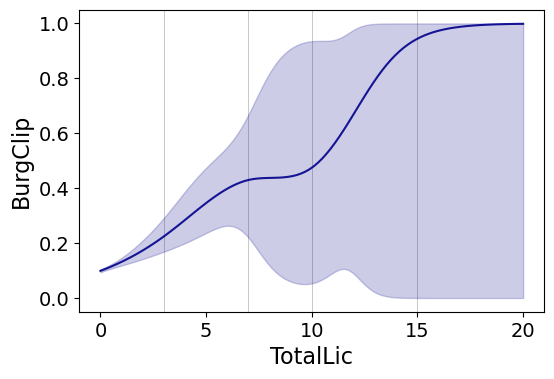I’m not sure how to do this in a way you can get prediction intervals (I know how to do it for Gaussian models, but not for the other glm families, prediction intervals probably don’t make sense for binomial data anyway). But one thing I could expand on in the future is to do quantile regression instead of glm models. # Smooth Plots by Group Sometimes you want to do the smoothed regression plots with interactions per groups. I have two helper functions to do this. One is group_rcs_plot. Here I use the good old iris data to illustrate, which I will explain why in a second. #Superimposing rcs on the same plot iris = sns.load_dataset('iris') smooth.group_rcs_plot(iris,'sepal_length','sepal_width', 'species',colors=None,num_knots=3)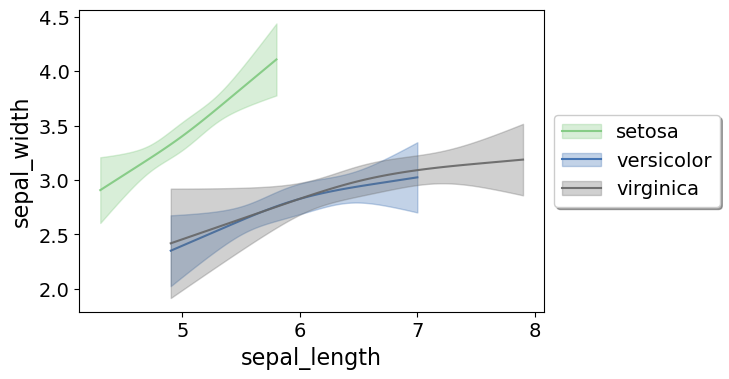If you pass in the num_knots argument, the knot locations are different for each subgroup of data (which I like as a default). If you pass in the knots argument and the locations, they are the same though for each subgroup. Note that the way I estimate the models here I estimate three different models on the subsetted data frame, I do not estimate a stacked model with group interactions. So the error bands will be a bit wider than estimating the stacked model. Sometimes superimposing many different groups is tough to visualize. So then a good option is to make a set of small multiple plots. To help with this, I’ve made a function loc_error, to pipe into seaborn’s small multiple set up: #Small multiple example g = sns.FacetGrid(iris, col='species',col_wrap=2) g.map_dataframe(smooth.loc_error, x='sepal_length', y='sepal_width', num_knots=3) g.set_axis_labels("Sepal Length", "Sepal Width")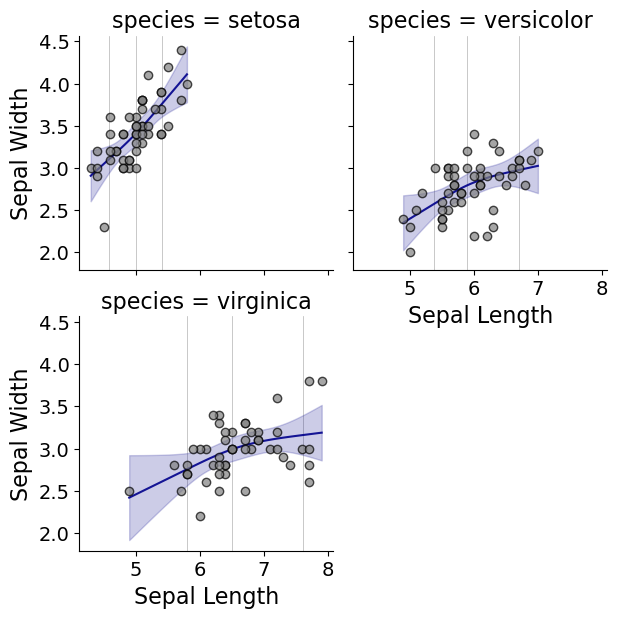And here you can see that the not locations are different for each subset, and this plot by default includes the original observations. # Using the Formula Interface for Plots Finally, I’ve been experimenting a bit with using the input in a formula interface, more similar to the way ggplot in R allows you to do this. So this is a new function, plot_form, and here is an example Poisson linear model: smooth.plot_form(data=DC_crime,x='TotalLic',y='TotalCrime', form='TotalCrime ~ TotalLic', fam=sm.families.Poisson(), marker_size=12)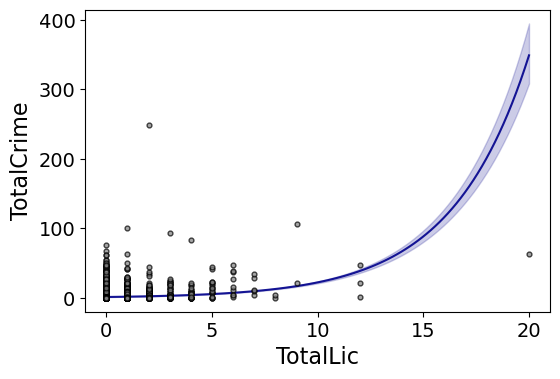You can see the explosive effect I talked about, which is common for Poisson/negative binomial models. Here with the formula interface you can do other things, such as a polynomial regression: #Can do polynomial terms smooth.plot_form(data=DC_crime,x='TotalLic',y='TotalCrime', form='TotalCrime ~ TotalLic + TotalLic**2 + TotalLic**3', fam=sm.families.Poisson(), marker_size=12) Which here ends up being almost indistinguishable from the linear terms. You can do other smoothers that are available in the patsy library as well, here are bsplines: #Can do other smoothers smooth.plot_form(data=DC_crime,x='TotalLic',y='TotalCrime', form='TotalCrime ~ bs(TotalLic,df=4,degree=3)', fam=sm.families.Poisson(), marker_size=12)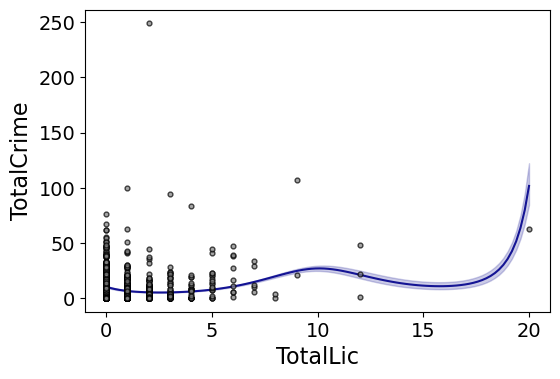I don’t really have a good reason to prefer restricted cubic splines to bsplines, I am just more familiar with restricted cubic splines (and this plot does not illustrate the knot locations that were by default chosen, although you could pass in knot locations to the bs function). You can also do other transformations of the x variable. So here if you take the square root of the total number of licenses helps with the explosive effect somewhat: #Can do transforms of the X variable smooth.plot_form(data=DC_crime,x='TotalLic',y='TotalCrime', form='TotalCrime ~ np.sqrt(TotalLic)', fam=sm.families.Poisson(), marker_size=12)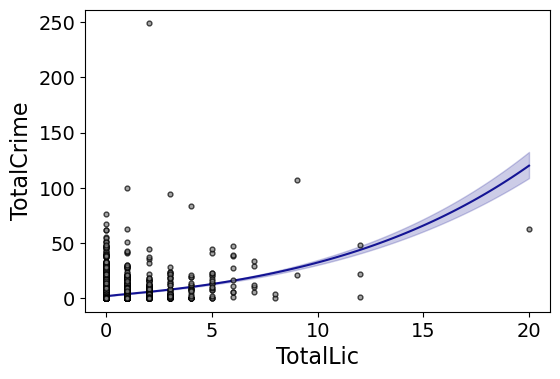In the prior blog post about explosive Poisson models I also showed a broken stick type model if you wanted to log the x variable but it has zero values. #Can do multiple transforms of the X variable smooth.plot_form(data=DC_crime,x='TotalLic',y='TotalCrime', form='TotalCrime ~ np.log(TotalLic.clip(1)) + I(TotalLic==0)', fam=sm.families.Poisson(), marker_size=12)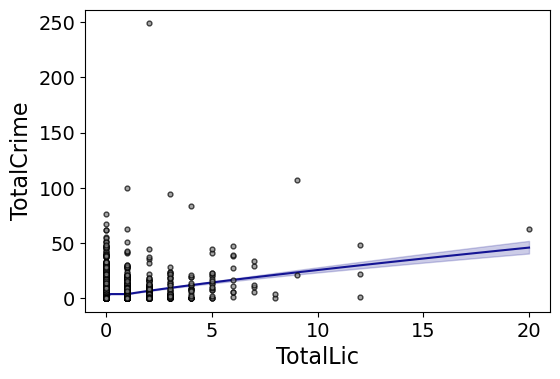Technically this “works” if you transform the Y variable as well, but the resulting plot is misleading, and the prediction interval is for the transformed variable. E.g. if you pass a formula 'np.log(TotalCrime+1) ~ TotalLic', you would need to exponentiate the the predictions and subtract 1 to get back to the original scale (and then the line won’t be the mean anymore, but the confidence intervals are OK). I will need to see if I can figure out patsy and sympy to be able to do the inverse transformation to even do that. That type of transform to the y variable directly probably only makes sense for linear models, and then I would also maybe need to do a Duan type smearing estimate to get the mean effect right. # Making aoristic density maps in R I saw Jerry the other day made/updated an R package to do aoristic analysis. A nice part of this is that it returns the weights breakdown for individual cases, which you can then make maps of. My goto hot spot map for data visualization, kernel density maps, are a bit tough to work with weighted data though in R (tough is maybe not the right word, to use ggplot it takes a bit of work leveraging other packages). So here are some notes on that. I have provided the data/code here. It is burglaries in Dallas, specifically I filter out just for business burglaries. # R Code Snippet First, for my front end I load the libraries I will be using, and change the working directory to where my data is located. ############################ library(aoristic) #aoristic analysis library(rgdal) #importing spatial data library(spatstat) #weighted kde library(raster) #manipulate raster object library(ggplot2) #for contour graphs library(sf) #easier to plot sf objects my_dir <- "D:\\Dropbox\\Dropbox\\Documents\\BLOG\\aoristic_maps_R\\data_analysis" setwd(my_dir) ############################ Next I just have one user defined function, this takes an input polygon (the polygon that defines the borders of Dallas here), and returns a raster grid covering the bounding box. It also have an extra data field, to say whether the grid cell is inside/outside of the boundary. (This is mostly convenient when creating an RTM style dataset to make all the features conform to the same grid cells.) ########################### #Data Manipulation Functions #B is border, g is size of grid cell on one side BaseRaster <- function(b,g){ base_raster <- raster(ext = extent(b), res=g) projection(base_raster) <- crs(b) mask_raster <- rasterize(b, base_raster, getCover=TRUE) #percentage of cover, 0 is outside return(mask_raster) } ########################### The next part I grab the datasets I will be using, a boundary file for Dallas (in which I chopped off the Lochs, so will not be doing an analysis of boat house burglaries today), and then the crime data. R I believe you always have to convert date-times when reading from a CSV (it never smartly infers that a column is date/time). And then I do some other data fiddling – Jerry has a nice function to check and make sure the date/times are all in order, and then I get rid of points outside of Dallas using the sp over function. Finally the dataset is for both residential/commercial, but I just look at the commercial burglaries here. ########################### #Get the datasets #Geo data boundary <- readOGR(dsn="Dallas_MainArea_Proj.shp",layer="Dallas_MainArea_Proj") base_Dallas <- BaseRaster(b=boundary,g=200) base_df <- as.data.frame(base_Dallas,long=TRUE,xy=TRUE) #Crime Data crime_dat <- read.csv('Burglary_Dallas.csv', stringsAsFactors=FALSE) #prepping time fields crime_datBeg <- as.POSIXct(crime_dat$StartingDateTime, format="%m/%d/%Y %H:%M:%OS") crime_dat$End <- as.POSIXct(crime_dat$EndingDateTime, format="%m/%d/%Y %H:%M:%OS") #cleaning up data aor_check <- aoristic.datacheck(crime_dat, 'XCoordinate', 'YCoordinate', 'Beg', 'End') coordinates(crime_dat) <- crime_dat[,c('XCoordinate', 'YCoordinate')] crs(crime_dat) <- crs(boundary) over_check <- over(crime_dat, boundary) keep_rows <- (aor_check$aoristic_datacheck == 0) & (!is.na(over_check$city)) crime_dat_clean <- crime_dat[keep_rows,] #only look at business burgs to make it go abit faster busi_burgs <- crime_dat_clean[ crime_dat_clean$UCROffense == 'BURGLARY-BUSINESS', ]
###########################

The next part preps the aoristic weights. First, the aoristic.df function is from Jerry’s aoristic package. It returns the weights broken down by 168 hours per day of the week. Here I then just collapse across the weekdays into the same hour, which to do that is simple, just add up the weights.

After that it is some more geographic data munging using the spatstat package to do the heavy lifting for the weighted kernel density estimate, and then stuffing the result back into another data frame. My bandwidth here, 3000 feet, is a bit large but makes nicer looking maps. If you do this smaller you will have a more bumpy and localized hot spots in the kernel density estimate.

###########################
#aoristic weights

#This takes like a minute
res_weights <- aoristic.df(busi_burgs@data, 'XCoordinate', 'YCoordinate', 'Beg', 'End')

#Binning into same hourly bins
for (i in 1:24){
cols <- (0:6*24)+i+5
lab <- paste0("Hour",i)
res_weights[,c(lab)] <- rowSums(res_weights[,cols])
}

#Prepping the spatstat junk I need
peval <- rasterToPoints(base_Dallas)[,1:2]
spWin <- as.owin(as.data.frame(peval))
sp_ppp <- as.ppp(res_weights[,c('x_lon','y_lat')],W=spWin) #spp point pattern object

#Creating a dataframe with all of the weighted KDE
Hour_Labs <- paste0("Hour",1:24)

for (h in Hour_Labs){
sp_den <- density.ppp(sp_ppp,weights=res_weights[,c(h)],
sigma=3000,
edge=FALSE,warnings=FALSE)
sp_dat <- as.data.frame(sp_den)
kd_raster <- rasterFromXYZ(sp_dat,res=res(base_Dallas),crs=crs(base_Dallas))
base_df[,c(h)] <- as.data.frame(kd_raster,long=TRUE)$value } ########################### If you are following along, you may be wondering why all the hassle? It is partly because I want to use ggplot to make maps, but for its geom_contour it does not except weights, so I need to do the data manipulation myself to supply ggplot the weighted data in the proper format. First I turn my Dallas boundary into a simple feature sf object, then I create my filled contour graph, supplying the regular grid X/Y and the Z values for the first Hour of the day (so between midnight and 1 am). ########################### #now making contour graphs dallas_sf <- st_as_sf(boundary) #A plot for one hour of the day hour1 <- ggplot() + geom_contour_filled(data=base_df, aes(x, y, z = Hour1), bins=9) + geom_sf(data=dallas_sf, fill=NA, color='black') + scale_fill_brewer(palette="Greens") + ggtitle(' Hour [0-1)') + theme_void() + theme(legend.position = "none") hour1 png('Hour1.png', height=5, width=5, units="in", res=1000, type="cairo") hour1 dev.off() ###########################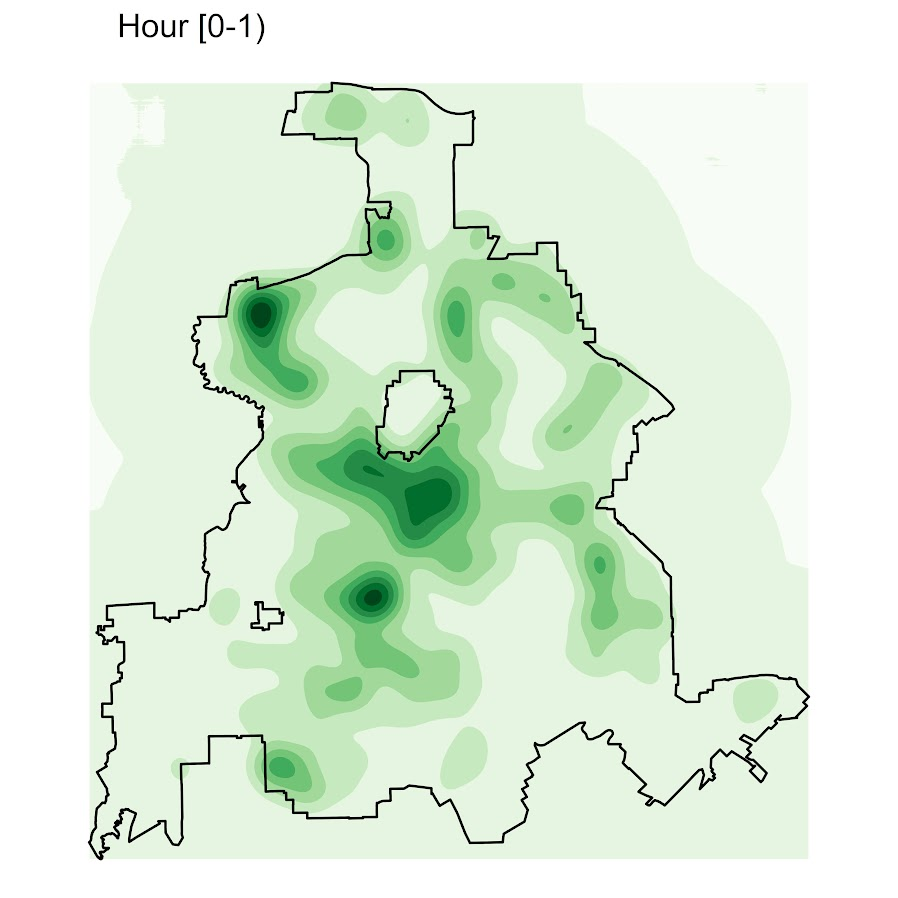Nice right! I have in the code my attempt to make a super snazzy small multiple plot, but that was not working out so well for me. But you can then go ahead and make up other slices if you want. Here is an example of taking an extended lunchtime time period. ########################### #Plot for the afternoon time period base_df$Afternoon <- rowSums(base_df[,paste0("Hour",10:17)])

afternoon <- ggplot() +
geom_contour_filled(data=base_df, aes(x, y, z = Afternoon), bins=9) +
geom_sf(data=dallas_sf, fill=NA, color='black') +
scale_fill_brewer(palette="Greens") +
ggtitle('       Hour [9:00-17:00)') +
theme_void() + theme(legend.position = "none")
afternoon
###########################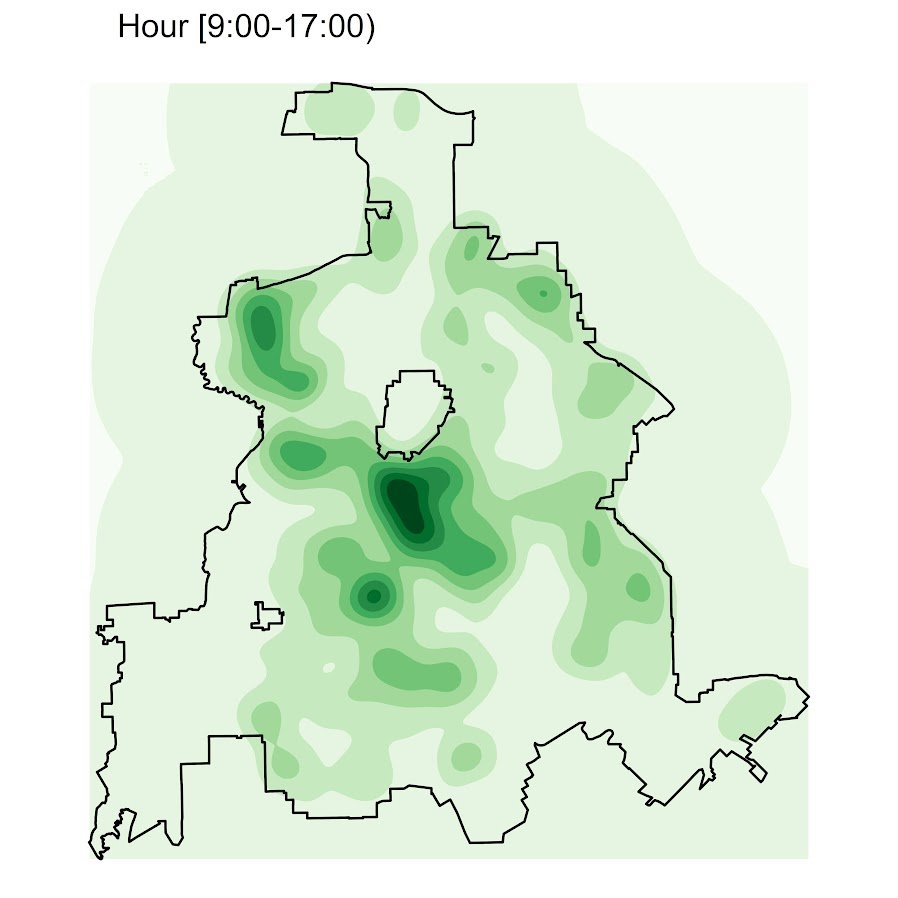So you can see that the patterns only slightly changed compared to the midnight prior graph.

Note that these plots will have different breaks, but you could set them to be equal by simply specifying a breaks argument in the geom_contour_filled call.

I will leave it up so someone who is more adept at R code than me to make a cool animated viz over time from this. But that is a way to mash up the temporal weights in a map.

# Notes on making scatterplots in matplotlib and seaborn

Many of my programming tips, like my notes for making Leaflet maps in R or margins plots in Stata, I’ve just accumulated doing projects over the years. My current workplace is a python shop though, so I am figuring it out all over for some of these things in python. I made some ugly scatterplots for a presentation the other day, and figured it would be time to spend alittle time making some notes on making them a bit nicer.

For prior python graphing post examples, I have:

For this post, I am going to use the same data I illustrated with SPSS previously, a set of crime rates in Appalachian counties. Here you can download the dataset and the python script to follow along.

# Making scatterplots using matplotlib

So first for the upfront junk, I load my libraries, change my directory, update my plot theme, and then load my data into a dataframe crime_dat. I technically do not use numpy in this script, but soon as I take it out I’m guaranteed to need to use np. for something!

################################################################
import pandas as pd
import numpy as np
import os
import matplotlib
import matplotlib.pyplot as plt
import seaborn as sns

my_dir = r'C:\Users\andre\OneDrive\Desktop\big_scatter'
os.chdir(my_dir)

andy_theme = {'axes.grid': True,
'grid.linestyle': '--',
'legend.framealpha': 1,
'legend.facecolor': 'white',
'legend.fontsize': 14,
'legend.title_fontsize': 16,
'xtick.labelsize': 14,
'ytick.labelsize': 14,
'axes.labelsize': 16,
'axes.titlesize': 20,
'figure.dpi': 100}

matplotlib.rcParams.update(andy_theme)
################################################################

First, lets start from the base scatterplot. After defining my figure and axis objects, I add on the ax.scatter by pointing the x and y’s to my pandas dataframe columns, here Burglary and Robbery rates per 100k. You could also instead of starting from the matplotlib objects start from the pandas dataframe methods (as I did in my prior histogram post). I don’t have a good reason for using one or the other.

Then I set the axis grid lines to be below my points (is there a way to set this as a default?), and then I set my X and Y axis labels to be nicer than the default names.

################################################################
#Default scatterplot
fig, ax = plt.subplots(figsize=(6,4))
ax.scatter(crime_dat['burg_rate'], crime_dat['rob_rate'])
ax.set_axisbelow(True)
ax.set_xlabel('Burglary Rate per 100,000')
ax.set_ylabel('Robbery Rate per 100,000')
plt.savefig('Scatter01.png', dpi=500, bbox_inches='tight')
plt.show()
################################################################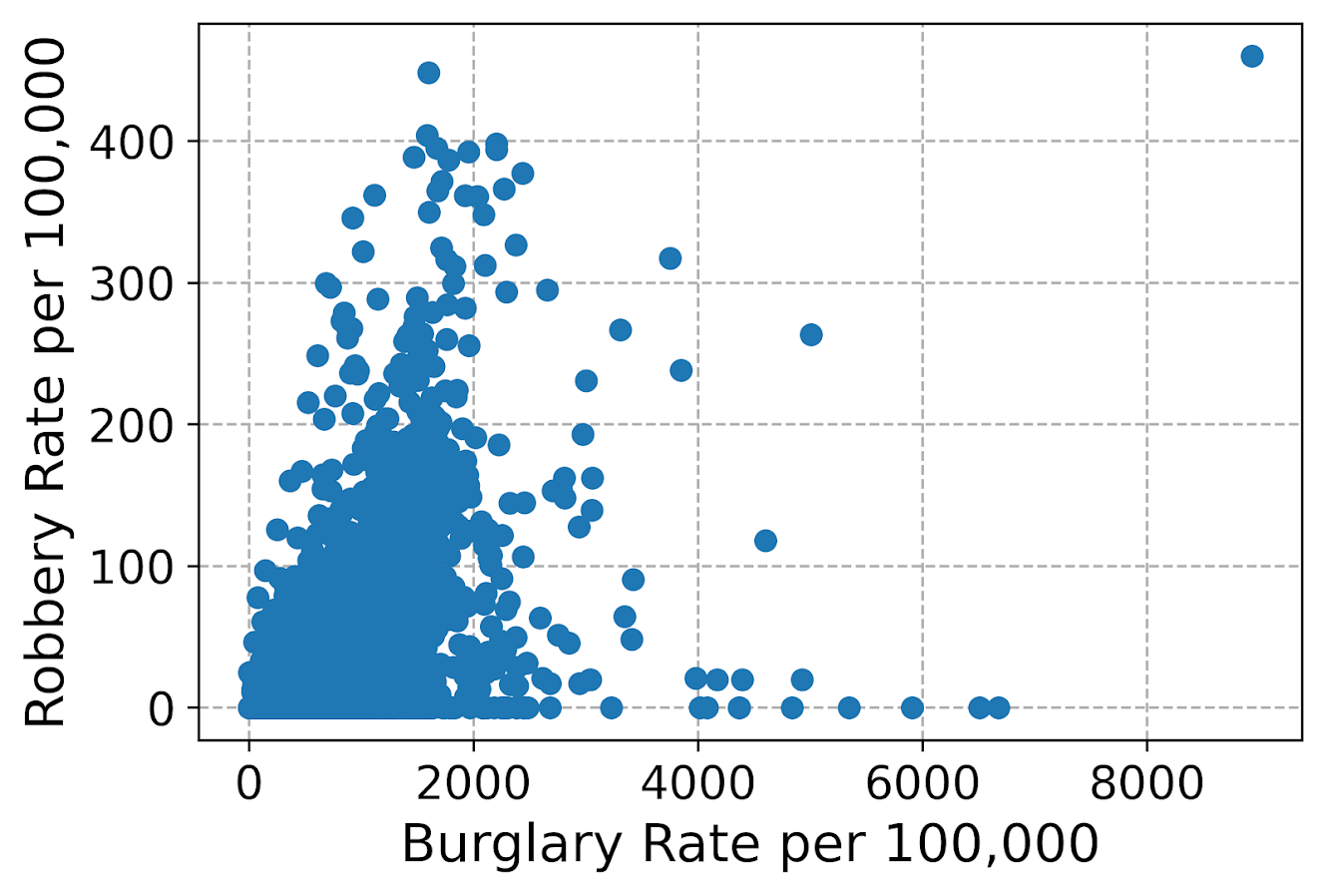You can see here the default point markers, just solid blue filled circles with no outline, when you get a very dense scatterplot just looks like a solid blob. I think a better default for scatterplots is to plot points with an outline. Here I also make the interior fill slightly transparent. All of this action is going on in the ax.scatter call, all of the other lines are the same.

################################################################
#Making points have an outline and interior fill
fig, ax = plt.subplots(figsize=(6,4))
ax.scatter(crime_dat['burg_rate'], crime_dat['rob_rate'],
c='grey', edgecolor='k', alpha=0.5)
ax.set_axisbelow(True)
ax.set_xlabel('Burglary Rate per 100,000')
ax.set_ylabel('Robbery Rate per 100,000')
plt.savefig('Scatter02.png', dpi=500, bbox_inches='tight')
plt.show()
################################################################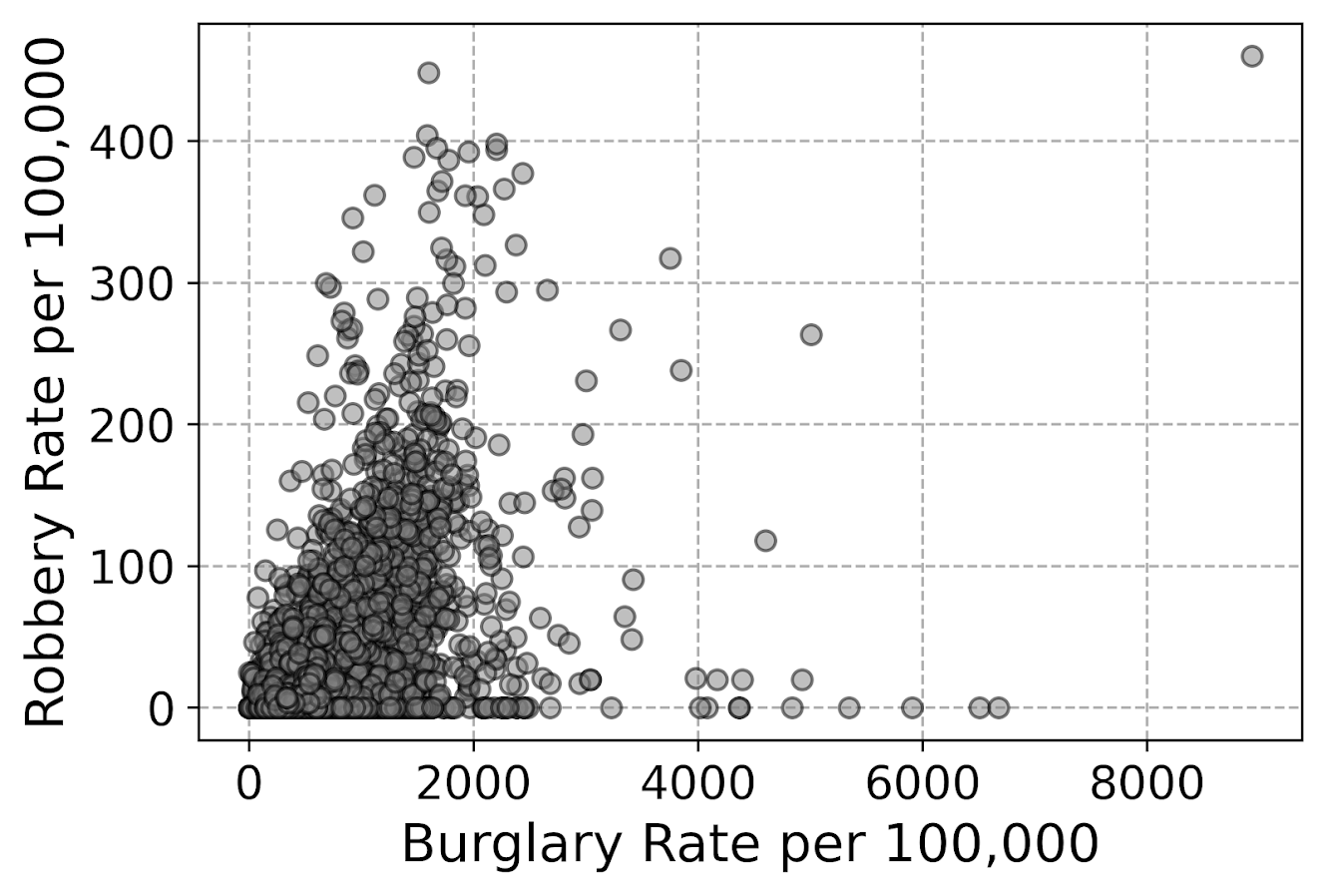So that is better, but we still have quite a bit of overplotting going on. Another quick trick is to make the points smaller and up the transparency by setting alpha to a lower value. This allows you to further visualize the density, but then makes it a bit harder to see individual points – if you started from here you might miss that outlier in the upper right.

Note I don’t set the edgecolor here, but if you want to make the edges semitransparent as well you could do edgecolor=(0.0, 0.0, 0.0, 0.5), where the last number of is the alpha transparency tuner.

################################################################
#Making the points small and semi-transparent
fig, ax = plt.subplots(figsize=(6,4))
ax.scatter(crime_dat['burg_rate'], crime_dat['rob_rate'], c='k',
alpha=0.1, s=4)
ax.set_axisbelow(True)
ax.set_xlabel('Burglary Rate per 100,000')
ax.set_ylabel('Robbery Rate per 100,000')
plt.savefig('Scatter03.png', dpi=500, bbox_inches='tight')
plt.show()
################################################################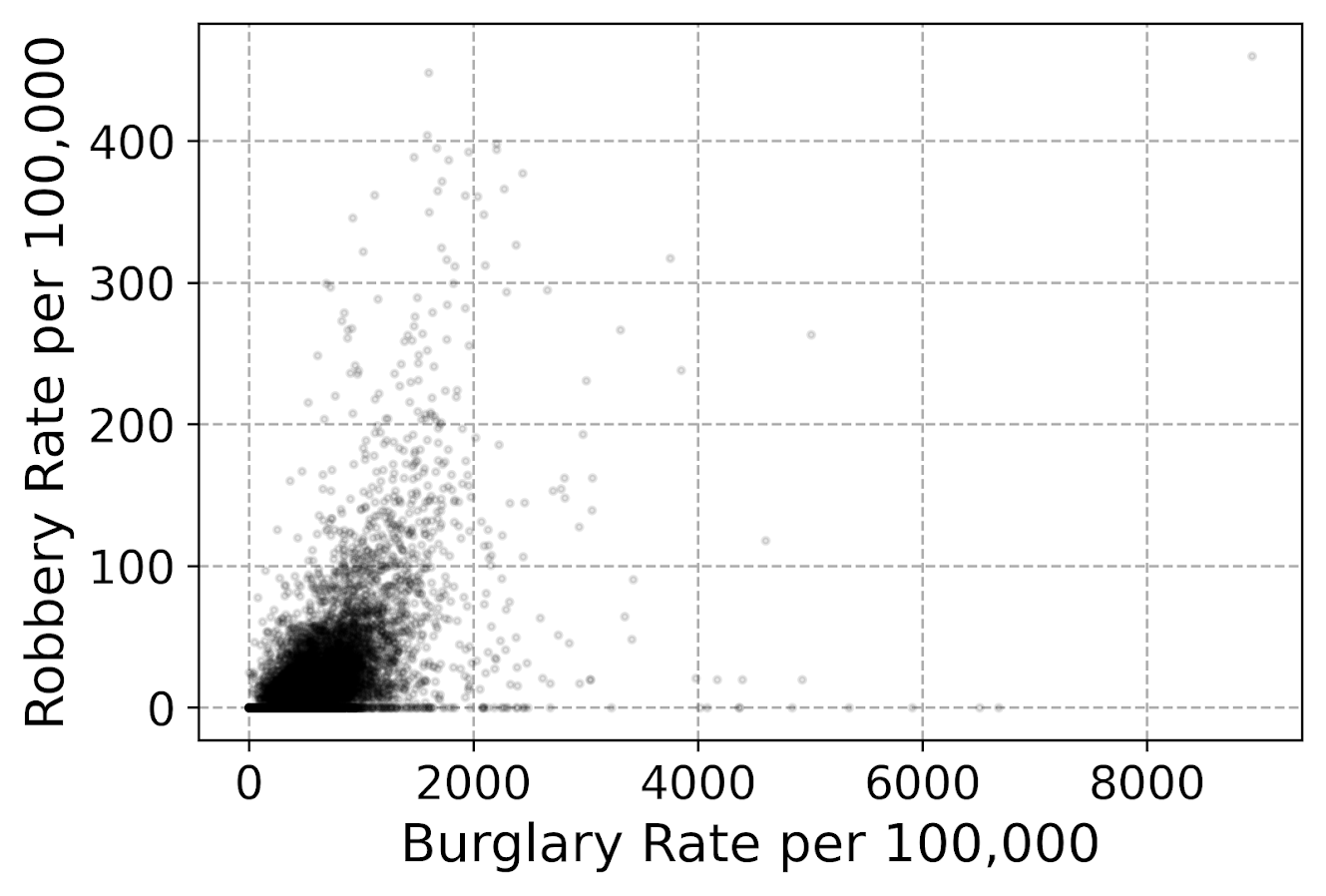This dataset has around 7.5k rows in it. For most datasets of anymore than a hundred points, you often have severe overplotting like you do here. One way to solve that problem is to bin observations, and then make a graph showing the counts within the bins. Matplotlib has a very nice hexbin method for doing this, which is easier to show than explain.

################################################################
#Making a hexbin plot
fig, ax = plt.subplots(figsize=(6,4))
hb = ax.hexbin(crime_dat['burg_rate'], crime_dat['rob_rate'],
gridsize=20, edgecolors='grey',
cmap='inferno', mincnt=1)
ax.set_axisbelow(True)
ax.set_xlabel('Burglary Rate per 100,000')
ax.set_ylabel('Robbery Rate per 100,000')
cb = fig.colorbar(hb, ax=ax)
plt.savefig('Scatter04.png', dpi=500, bbox_inches='tight')
plt.show()
################################################################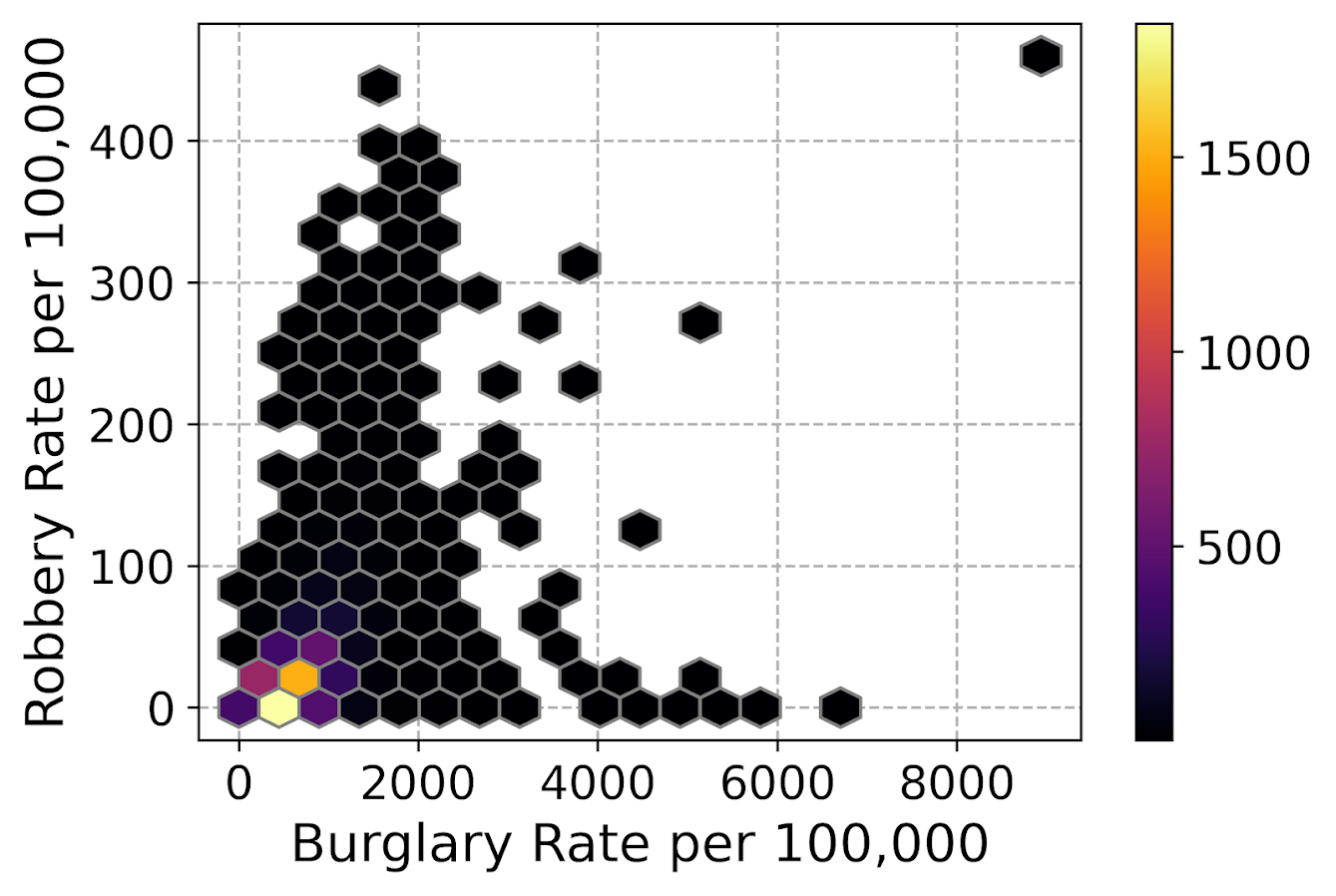So for the hexbins I like using the mincnt=1 option, as it clearly shows areas with no points, but then you can still spot the outliers fairly easy. (Using white for the edge colors looks nice as well.)

You may be asking, what is up with that outlier in the top right? It ends up being Letcher county in Kentucky in 1983, which had a UCR population estimate of only 1522, but had a total of 136 burglaries and 7 robberies. This could technically be correct (only some local one cop town reported, and that town does not cover the whole county), but I’m wondering if this is a UCR reporting snafu.

It is also a good use case for funnel charts. I debated on making some notes here about putting in text labels, but will hold off for now. You can add in text by using ax.annotate fairly easy by hand, but it is hard to automate text label positions. It is maybe easier to make interactive graphs and have a tooltip, but that will need to be another blog post as well.

# Making scatterplots using seaborn

The further examples I show are using the seaborn library, imported earlier as sns. I like using seaborn to make small multiple plots, but it also has a very nice 2d kernel density contour plot method I am showing off.

Note this does something fundamentally different than the prior hexbin chart, it creates a density estimate. Here it looks pretty but creates a density estimate in areas that are not possible, negative crime rates. (There are ways to prevent this, such as estimating the KDE on a transformed scale and retransforming back, or reflecting the density back inside the plot would probably make more sense here, ala edge weighting in spatial statistics.)

Here the only other things to note are used filled contours instead of just the lines, I also drop the lowest shaded area (I wish I could just drop areas of zero density, note dropping the lowest area drops my outlier in the top right). Also I have a tough go of using the default bandwidth estimators, so I input my own.

################################################################
#Making a contour plot using seaborn
g = sns.kdeplot(crime_dat['burg_rate'], crime_dat['rob_rate'],
g.set_axisbelow(True)
g.set_xlabel('Burglary Rate per 100,000')
g.set_ylabel('Robbery Rate per 100,000')
plt.savefig('Scatter05.png', dpi=500, bbox_inches='tight')
plt.show()
################################################################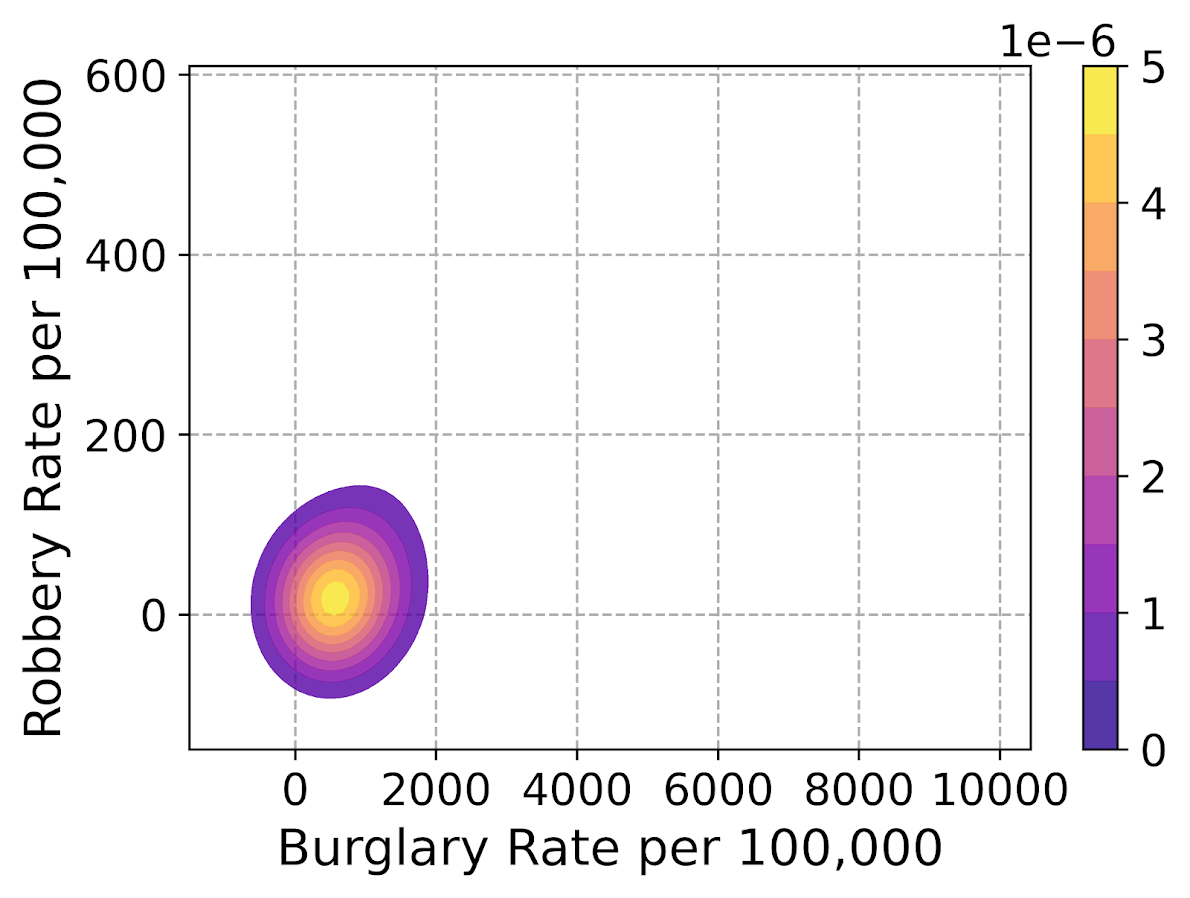So far I have not talked about the actual marker types. It is very difficult to visualize different markers in a scatterplot unless they are clearly separated. So although it works out OK for the Iris dataset because it is small N and the species are clearly separated, in real life datasets it tends to be much messier.

So I very rarely use multiple point types to symbolize different groups in a scatterplot, but prefer to use small multiple graphs. Here is an example of turning my original scatterplot, but differentiating between different county areas in the dataset. It is a pretty straightforward update using sns.FacetGrid to define the group, and then using g.map. (There is probably a smarter way to set the grid lines below the points for each subplot than the loop.)

################################################################
#Making a small multiple scatterplot using seaborn
g = sns.FacetGrid(data=crime_dat, col='subrgn',
col_wrap=2, despine=False, height=4)
g.map(plt.scatter, 'burg_rate', 'rob_rate', color='grey',
s=12, edgecolor='k', alpha=0.5)
g.set_titles("{col_name}")
for a in g.axes:
a.set_axisbelow(True)
g.set_xlabels('Burglary Rate per 100,000')
g.set_ylabels('Robbery Rate per 100,000')
plt.savefig('Scatter06.png', dpi=500, bbox_inches='tight')
plt.show()
################################################################And then finally I show an example of making a small multiple hexbin plot. It is alittle tricky, but this is an example in the seaborn docs of writing your own sub-plot function and passing that.

To make this work, you need to pass an extent for each subplot (so the hexagons are not expanded/shrunk in any particular subplot). You also need to pass a vmin/vmax argument, so the color scales are consistent for each subplot. Then finally to add in the color bar I just fiddled with adding an axes. (Again there is probably a smarter way to scoop up the plot coordinates for the last plot, but here I just experimented till it looked about right.)

################################################################
#Making a small multiple hexbin plot using seaborn

#https://stackoverflow.com/a/31385996/604456
def loc_hexbin(x, y, **kwargs):
kwargs.pop("color", None)
plt.hexbin(x, y, gridsize=20, edgecolor='grey',
cmap='inferno', mincnt=1,
vmin=1, vmax=700, **kwargs)

g = sns.FacetGrid(data=crime_dat, col='subrgn',
col_wrap=2, despine=False, height=4)
g.map(loc_hexbin, 'burg_rate', 'rob_rate',
edgecolors='grey', extent=[0, 9000, 0, 500])
g.set_titles("{col_name}")
for a in g.axes:
a.set_axisbelow(True)
#This goes x,y,width,height
cax = g.fig.add_axes([0.55, 0.09, 0.03, .384])
plt.colorbar(cax=cax, ax=g.axes)
g.set_xlabels('Burglary Rate per 100,000')
g.set_ylabels('Robbery Rate per 100,000')
plt.savefig('Scatter07.png', dpi=500, bbox_inches='tight')
plt.show()
################################################################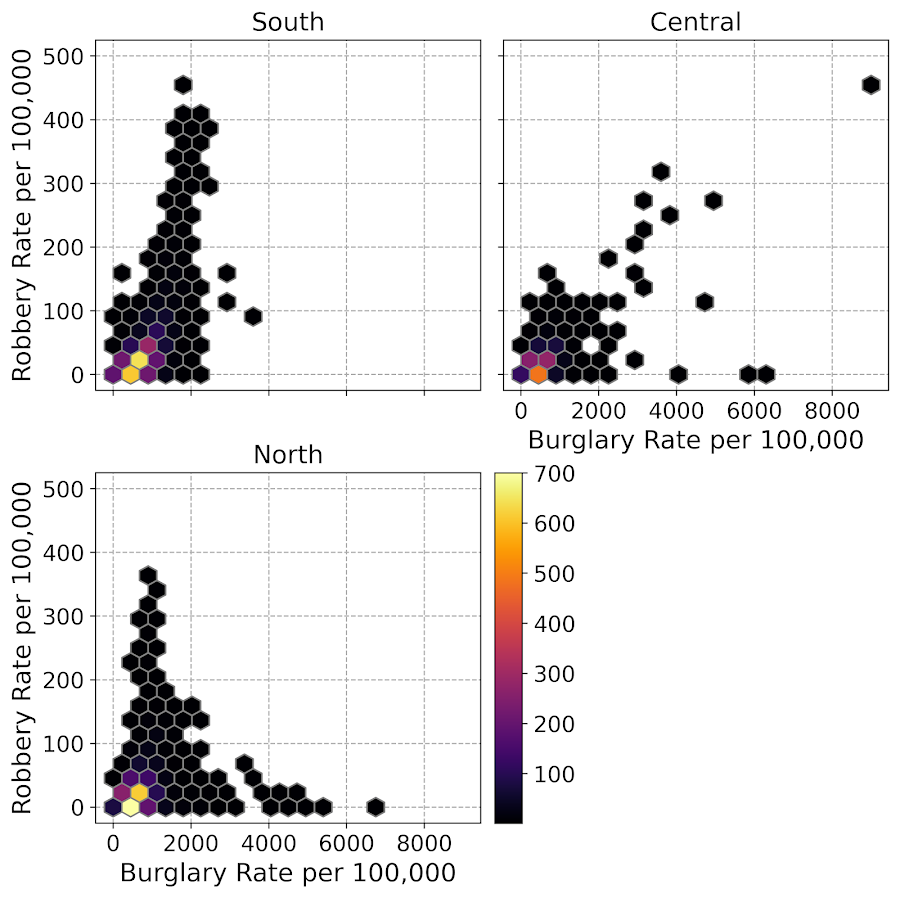Another common task with scatterplots is to visualize a smoother, e.g. E[Y|X] the expected mean of Y conditional on X, or you could do any other quantile, etc. That will have to be another post though, but for examples I have written about previously I have jittering 0/1 data, and visually weighted regression.

# Notes on making Leaflet maps in R

The other day I wrote a blog post for crimrxiv about posting interactive graphics on their pre-print sharing service. I figured it would be good to share my notes on making interactive maps, and to date I’ve mostly created these using the R leaflet library.

The reason I like these interactive maps is they allow you to zoom in and look at hot spots of crime. With the slippy base maps you can then see, oh OK this hot spot is by a train station, or an apartment complex, etc. It also allows you to check out specific data labels via pop-ups as I will show.

I’m using data from my paper on creating cost of crime weighted hot spots in Dallas (that will be forthcoming in Police Quarterly soonish). But I have posted a more direct set of replicating code for the blog post here.

# R Code

So first for the R libraries I am using, I also change the working directory to where I have my data located on my Windows machine.

##########################################################
#This code creates a nice leaflet map of my DBSCAN areas

library(sp)          #spatial objects
library(leaflet)     #for creating interactive maps
library(htmlwidgets) #for exporting interactive maps

#will need to change baseLoc if replicating on your machine
baseLoc <- "D:\\Dropbox\\Dropbox\\Documents\\BLOG\\leaflet_R_examples\\Analysis"
setwd(baseLoc)
##########################################################

Second, I read in my shapefiles using the rgdal library. This is important, as it includes the projection information. To plot the spatial objects on a slippy map they need to be in the Web Mercator projection (or technically no projection, just a coordinate reference system for the globe). As another trick I like with these basemaps, for the outlined area (the Dallas boundary here), it is easier to plot as a line spatial object, as opposed to an empty filled polygon. You don’t need to worry about the order of the layers as much that way.

##########################################################
#Get the boundary data and DBSCAN data

#Now convert to WGS
DalLatLon <- spTransform(boundary,CRS("+init=epsg:4326"))
DallLine <- as(DalLatLon, 'SpatialLines') #Leaflet useful for boundaries to be lines instead of areas
dbscan_LatLon <- spTransform(dbscan_areas,CRS("+init=epsg:4326") )

#Quick and Dirty plot to check projections are OK
plot(DallLine)
##########################################################

Next part, I have a custom function I have made to make pop-up labels for these leaflet maps. First I need to read in a table with the data info for the hot spot areas and merge that into the spatial object. Then the way my custom function works is I pass it the dataset, then I have arguments for the variables I want, and the way I want them labeled. The function does the work of making the labels bolded and putting in line breaks into the HTML. (No doubt others have created nice libraries to do HTML tables/graphs inside the pop-ups that I am unaware of.) If you check out the final print statement, it shows the HTML it built for one of the labels, <strong>ID: </strong>1<br><strong>$(Thousands): </strong>116.9<br><strong>PAI: </strong>10.3<br><strong>Street Length (Miles): </strong>0.4 ########################################################## #Function for labels #read in data crime_stats <- read.csv('ClusterStats_wlen.csv', stringsAsFactors=FALSE) dbscan_stats <- crime_stats[crime_stats$type == 'DBSCAN',]
dbscan_stats$clus_id <- as.numeric(dbscan_stats$AreaStr) #because factors=False!

#merge into the dbscan areas
dbscan_LL <- merge(dbscan_LatLon,dbscan_stats)

LabFunct <- function(data,vars,labs){
n <- length(labs)
for (i in 2:n){
}
}

#create labels
vs <- c('AreaStr', 'val_th', 'PAI_valth_len', 'LenMile')
#Lazy, so just going to round these values
for (v in vs[-1]){
dbscan_LL@data[,v] <- round(dbscan_LL@data[,v],1)
}
lb <- c('ID: ','$(Thousands): ','PAI: ','Street Length (Miles): ') diss_lab <- LabFunct(dbscan_LL@data, vs, lb) print(diss_lab) #showing off just one ########################################################## Now finally onto the hotspot map. This is a bit to chew over, so I will go through bit-by-bit. ########################################################## HotSpotMap <- leaflet() %>% addProviderTiles(providers$OpenStreetMap, group = "Open Street Map") %>%
addProviderTiles(providers$CartoDB.Positron, group = "CartoDB Lite") %>% addPolylines(data=DallLine, color='black', weight=4, group="Dallas Boundary") %>% addPolygons(data=dbscan_LL,color = "blue", weight = 2, opacity = 1.0, fillOpacity = 0.5, group="DBSCAN Areas",popup=diss_lab, highlight = highlightOptions(weight = 5,bringToFront = TRUE)) %>% addLayersControl(baseGroups = c("Open Street Map","CartoDB Lite"), overlayGroups = c("Dallas Boundary","DBSCAN Areas"), options = layersControlOptions(collapsed = FALSE)) %>% addScaleBar(position = "bottomleft", options = scaleBarOptions(maxWidth = 100, imperial = TRUE, updateWhenIdle = TRUE)) HotSpotMap #this lets you view interactively #or save to a HTML file to embed in webpage saveWidget(HotSpotMap,"HotSpotMap.html", selfcontained = TRUE) ########################################################## First I create the empty leaflet() object. Because I am superimposing multiple spatial layers, I don’t worry about setting the default spatial layer. Second, I add in two basemap providers, OpenStreetMap and the grey scale CartoDB positron. Positron is better IMO for visualizing global data patterns, but the open street map is better for when you zoom in and want to see exactly what is around a hot spot area. Note when adding in a layer, I give it a group name. This allows you to later toggle which provider you want via a basegroup in the layers control. Next I add in the two spatial layers, the Dallas Boundary lines and then the hot spots. For the DBSCAN hot spots, I include a pop-up diss_lab for the dbscan hot spot layer. This allows you to click on the polygon, and you get the info I stuffed into that label vector earlier. The HTML is to make it print nicely. Finally then I add in a layers control, so you can toggle layers on/off. Basegroups mean that only one of the options can be selected, it doesn’t make sense to have multiple basemaps selected. Overlay you can toggle on/off as needed. Here the overlay doesn’t matter much due to the nature of the map, but if you have many layers (e.g. a hot spot map and a choropleth map of demographics) being able to toggle the layers on/off helps a bit more. Then as a final touch I add in a scale bar (that automatically updates depending on the zoom level). These aren’t my favorite with slippy maps, as I’m not even 100% sure what location the scale bar refers to offhand (the center of the map? Or literally where the scale bar is located?) But when zoomed into smaller areas like a city I guess it is not misleading. Here is a screenshot of this created map zoomed out to the whole city using the Positron grey scale base map. So it is tough to visualize the distribution of hot spots from this. If I wanted to do that in a static map I would likely just plot the hot spot centroids, and then make the circles bigger for areas that capture more crime.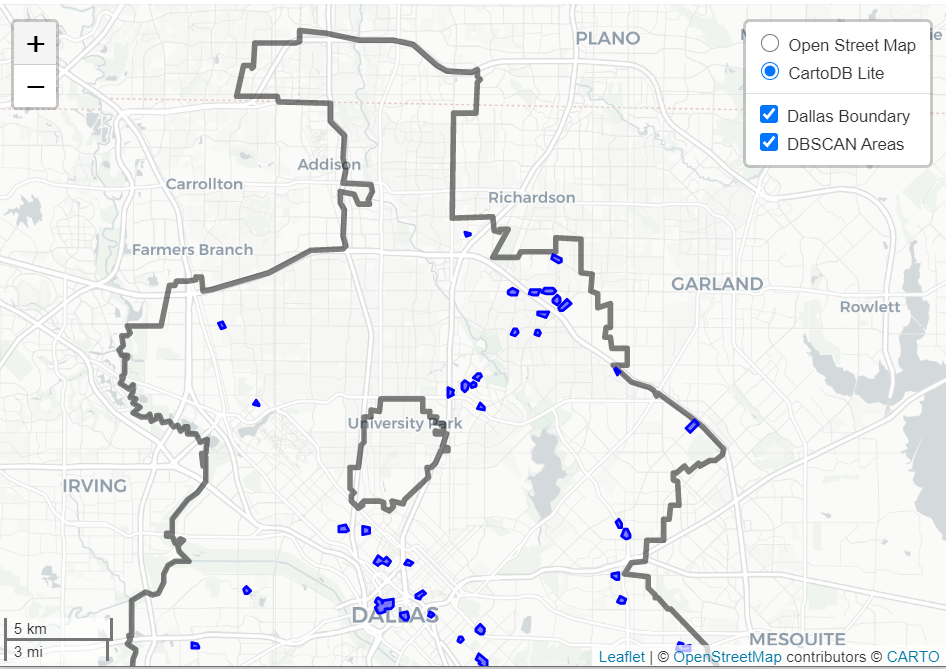But since we can zoom in, here is another screenshot zoomed in using the OpenStreetMap basemap, and also illustrating what my pop-up labels look like.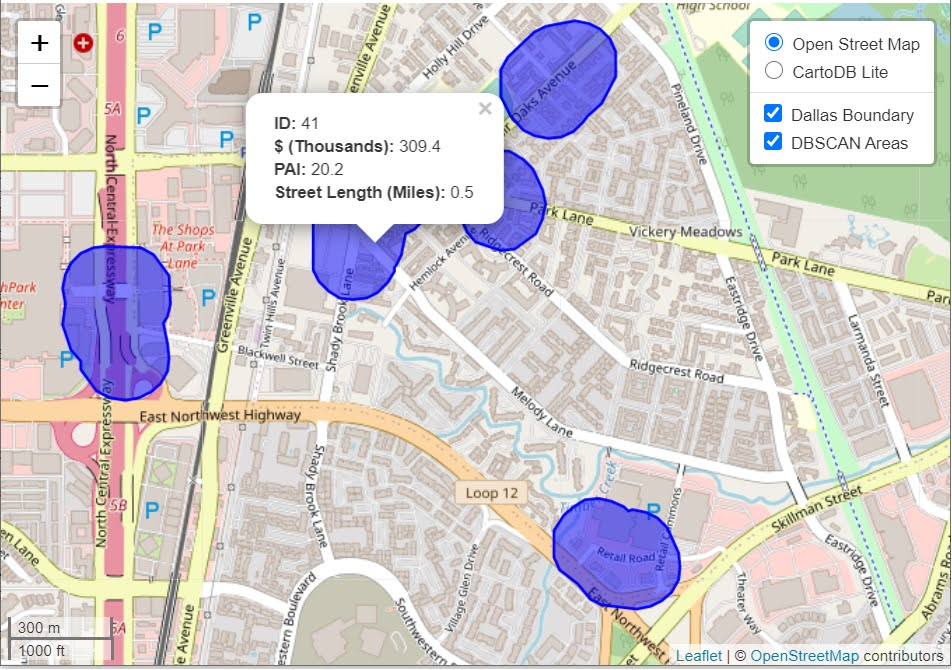I’m too lazy to post this exact map, but it is very similar to one I posted for my actual hot spots paper if you want to check it out directly. I host it on GitHub for free. Here I did not show how to make a choropleth map, but Jacob Kaplan in his R book has a nice example of that. And in the future I will have to update this to show how to do the same thing in python using the Folium library. I used Folium in this blog post if you want to dig into an example though for now. # Some more examples For some other examples of what is possible in Leaflet maps in R, here are some examples I made for my undergrad Communities and Crime class. I had students submit prediction assignments (e.g. predict the neighborhood with the most crime in Dallas, predict the street segment in Oak Cliff with the most violent crime, predict the bar with the most crimes nearby, etc.) I would then show the class the results, as well as where other students predicted. So here are some screen shots of those maps. Choropleth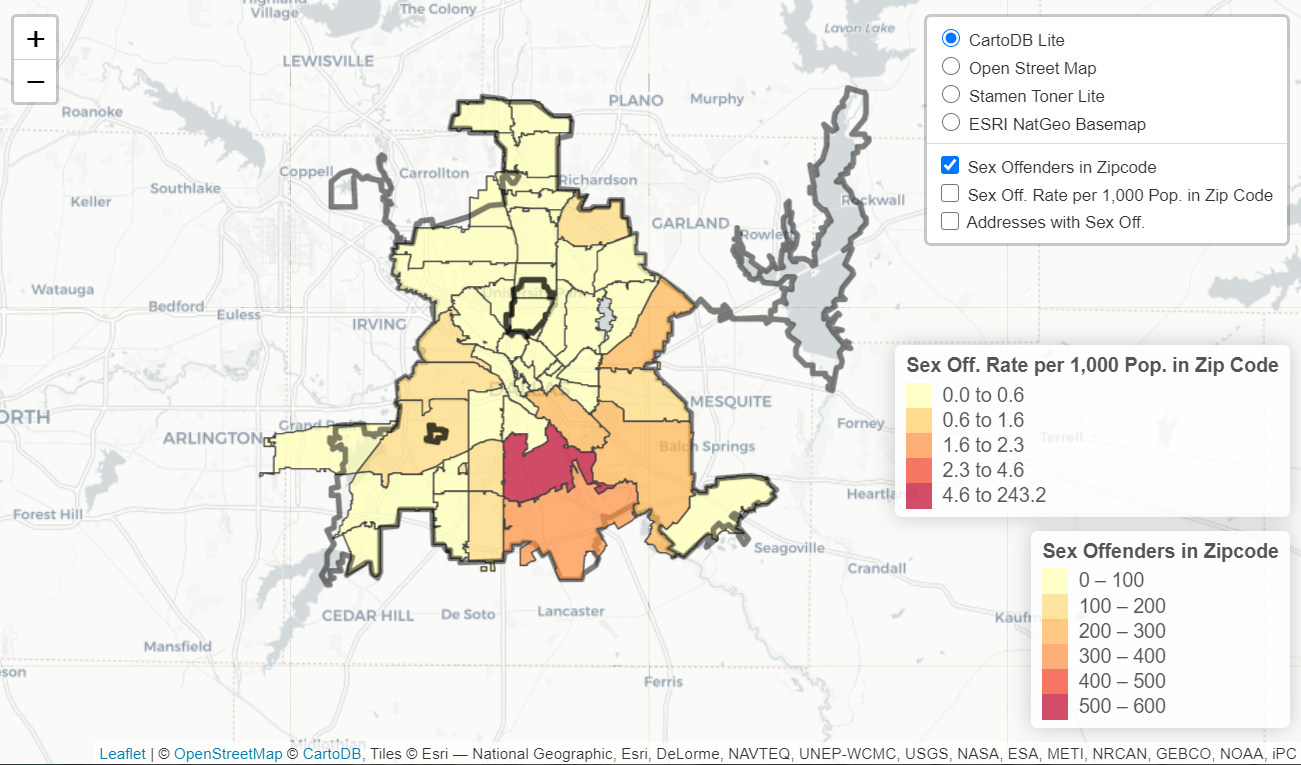Graduated Points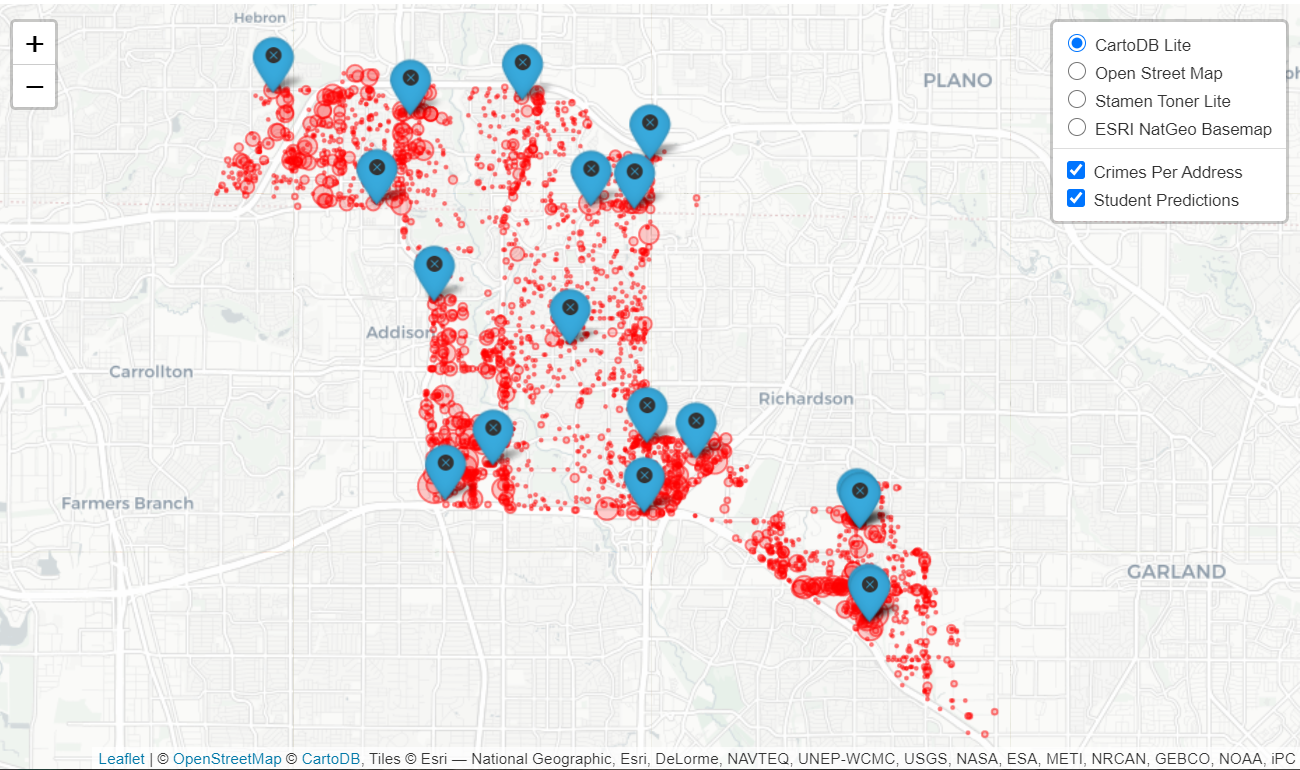Street Segment Viz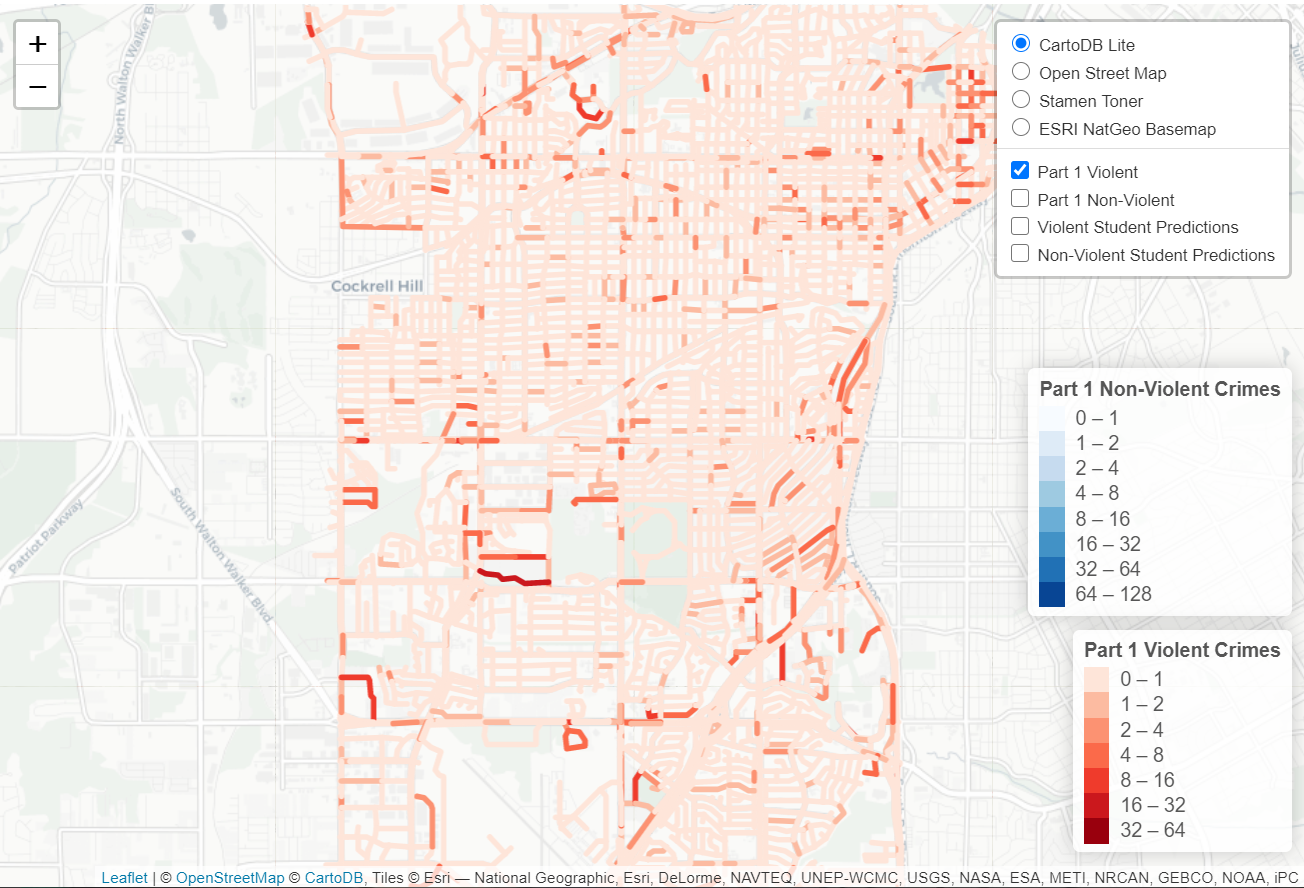# Using Steiner trees to select a subgraph of interest This is just a quick blog post. A crime analyst friend the other day posed a network problem to me. They had a social network in which they had particular individuals of interest, and wanted to show just a subset of that graph that connected those key individuals. The motivation was for plotting – if you show the entire hairball it can become really difficult to uncover any relationships. Here is an example gang network from this paper. I randomly chose 10 nodes to highlight (larger red circles), and you can see it is quite hairy. You often want to label the nodes for these types of graphs, but that becomes impossible with so many intertwined nodes.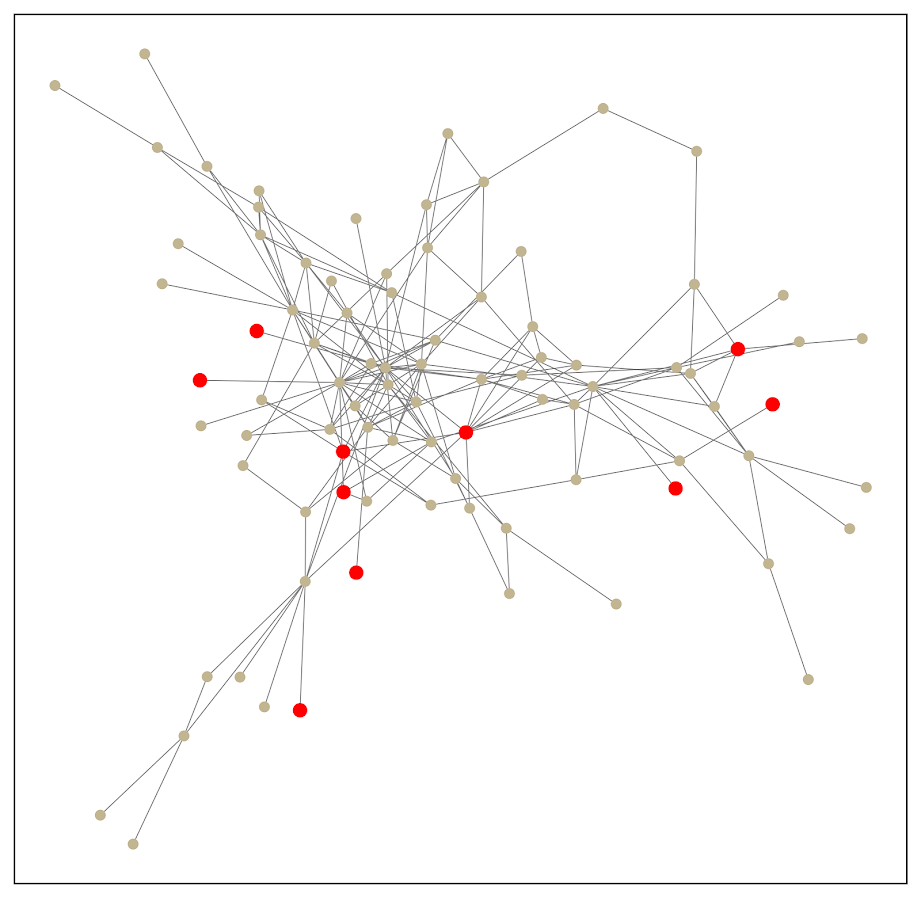One solution to select out a subgraph of the connected bits is to use a Steiner tree. Here is that graph after running the approximate Steiner tree algorithm in networkx (in python).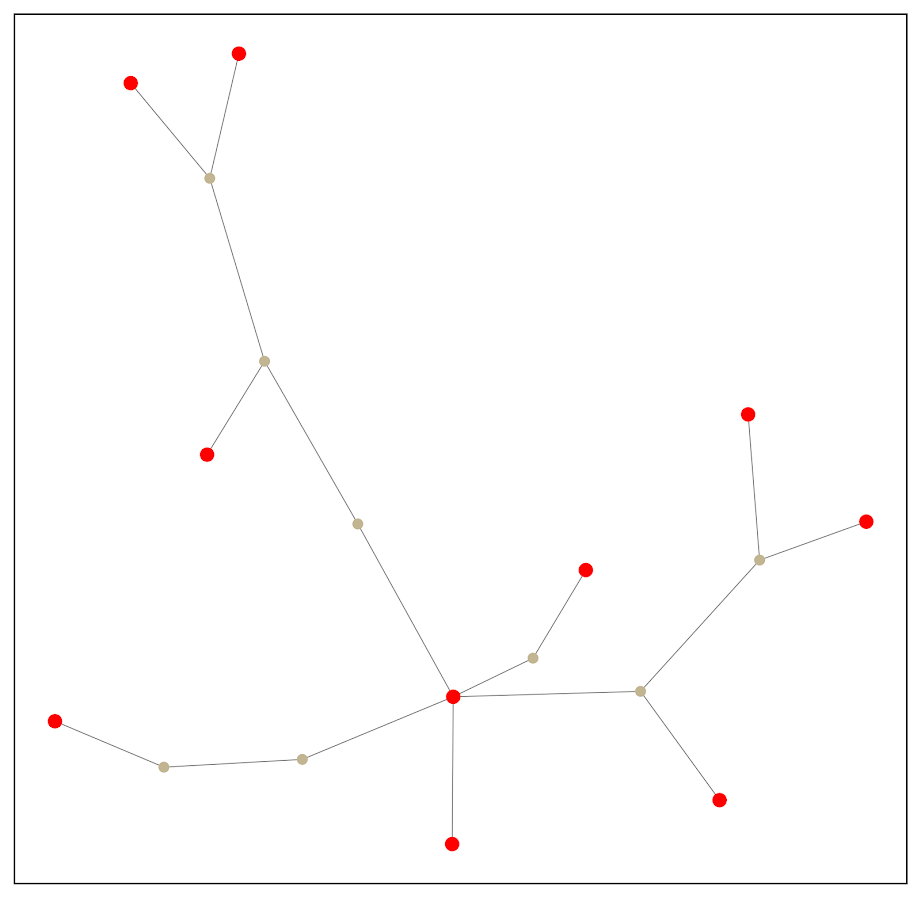Much simpler! And much more space to put additional labels. I’ve posted the code and data to replicate here. Initially I debated on solving this via setting up the problem as a min-cost-flow, where one of the highlighted nodes had the supply, and the other highlighted nodes had the demand. But this approximate algorithm in my few tests looks really good in selecting tiny subsets, so not much need. A few things to note about this. It is likely for many dense networks there will be many alternative subsets that are the same size, but different nodes (e.g. you can swap out a node and have the same looking network). A better approach to see connections between interesting nodes may be a betweenness centrality metric, where you only consider the flows between the highlighted nodes. A partial solution to that problem is to add nodes/edges back in after the Steiner tree subset. Here is an example where I add back in all first degree nodes to the red nodes of interest: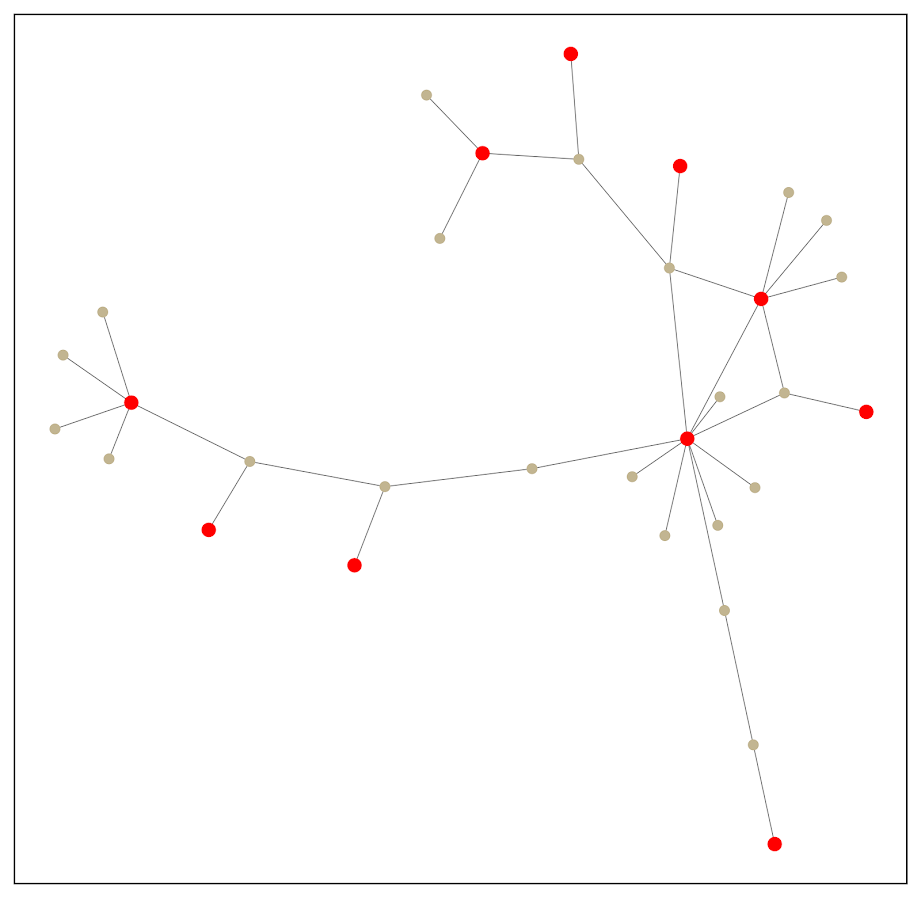So it is still a tiny enough network to plot. This just provides a way to identify higher order nodes of interest that aren’t directly connected to those red nodes. # Histogram notes in python with pandas and matplotlib Here are some notes (for myself!) about how to format histograms in python using pandas and matplotlib. The defaults are no doubt ugly, but here are some pointers to simple changes to formatting to make them more presentation ready. First, here are the libraries I am going to be using. import pandas as pd import numpy as np import matplotlib from matplotlib import pyplot as plt from matplotlib.ticker import StrMethodFormatter from matplotlib.ticker import FuncFormatter Then I create some fake log-normal data and three groups of unequal size. #generate three groups of differing size n = 50000 group = pd.Series(np.random.choice(3, n, p=[0.6, 0.35, 0.05])) #generate log-normal data vals = pd.Series(np.random.lognormal(mean=(group+2)*2,sigma=1)) dat = pd.concat([group,vals], axis=1) dat.columns = ['group','vals'] And note I change my default plot style as well. So if you are following along your plots may look slightly different than mine. One trick I like is using groupby and describe to do a simple textual summary of groups. But I also like transposing that summary to make it a bit nicer to print out in long format. (I use spyder more frequently than notebooks, so it often cuts off the output.) I also show setting the pandas options to a print format with no decimals. #Using describe per group pd.set_option('display.float_format', '{:,.0f}'.format) print( dat.groupby('group')['vals'].describe().T )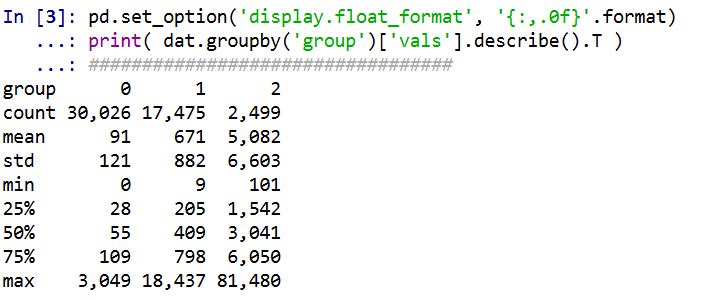Now onto histograms. Pandas has many convenience functions for plotting, and I typically do my histograms by simply upping the default number of bins. dat['vals'].hist(bins=100, alpha=0.8)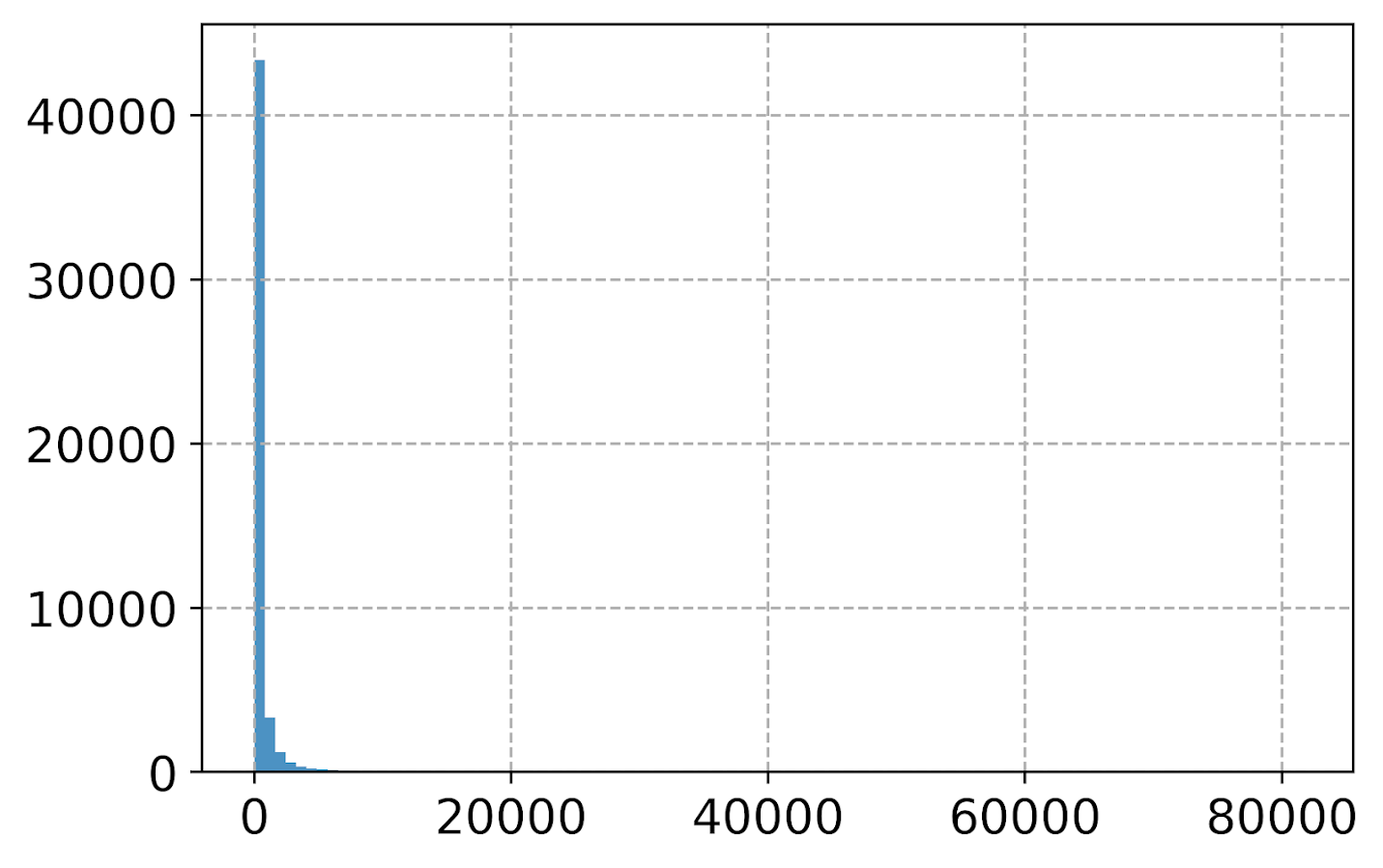Well that is not helpful! So typically when I see this I do a log transform. (Although note if you are working with low count data that can have zeroes, a square root transformation may make more sense. If you have only a handful of zeroes you may just want to do something like np.log([dat['x'].clip(1)) just to make a plot on the log scale, or some other negative value to make those zeroes stand out.) #Histogram On the log scale dat['log_vals'] = np.log(dat['vals']) dat['log_vals'].hist(bins=100, alpha=0.8)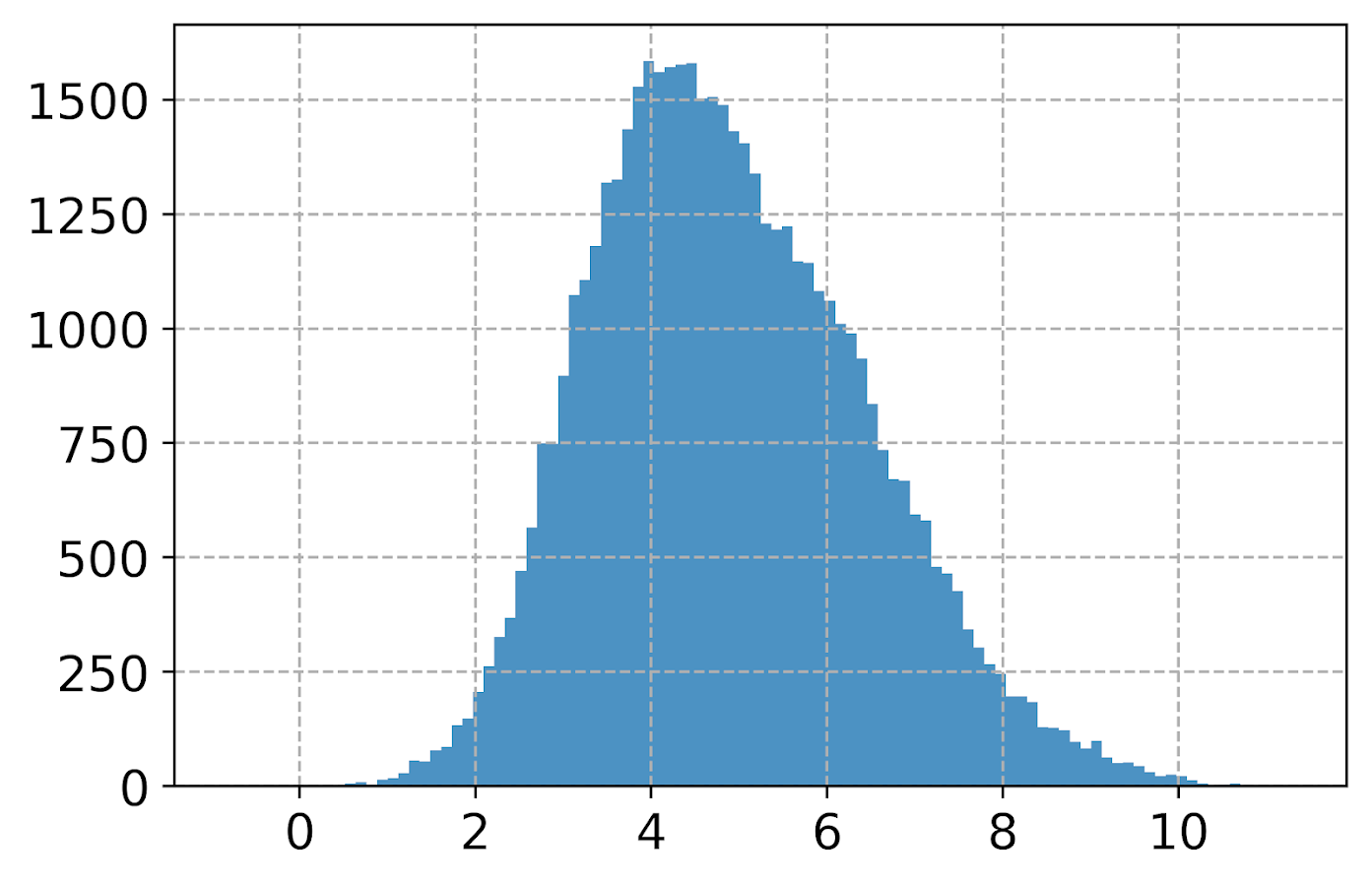Much better! It may not be obvious, but using pandas convenience plotting functions is very similar to just calling things like ax.plot or plt.scatter etc. So you can assign the plot to an axes object, and then do subsequent manipulations. (Don’t ask me when you should be putzing with axes objects vs plt objects, I’m just muddling my way through.) So here is an example of adding in an X label and title. #Can add in all the usual goodies ax = dat['log_vals'].hist(bins=100, alpha=0.8) plt.title('Histogram on Log Scale') ax.set_xlabel('Logged Values')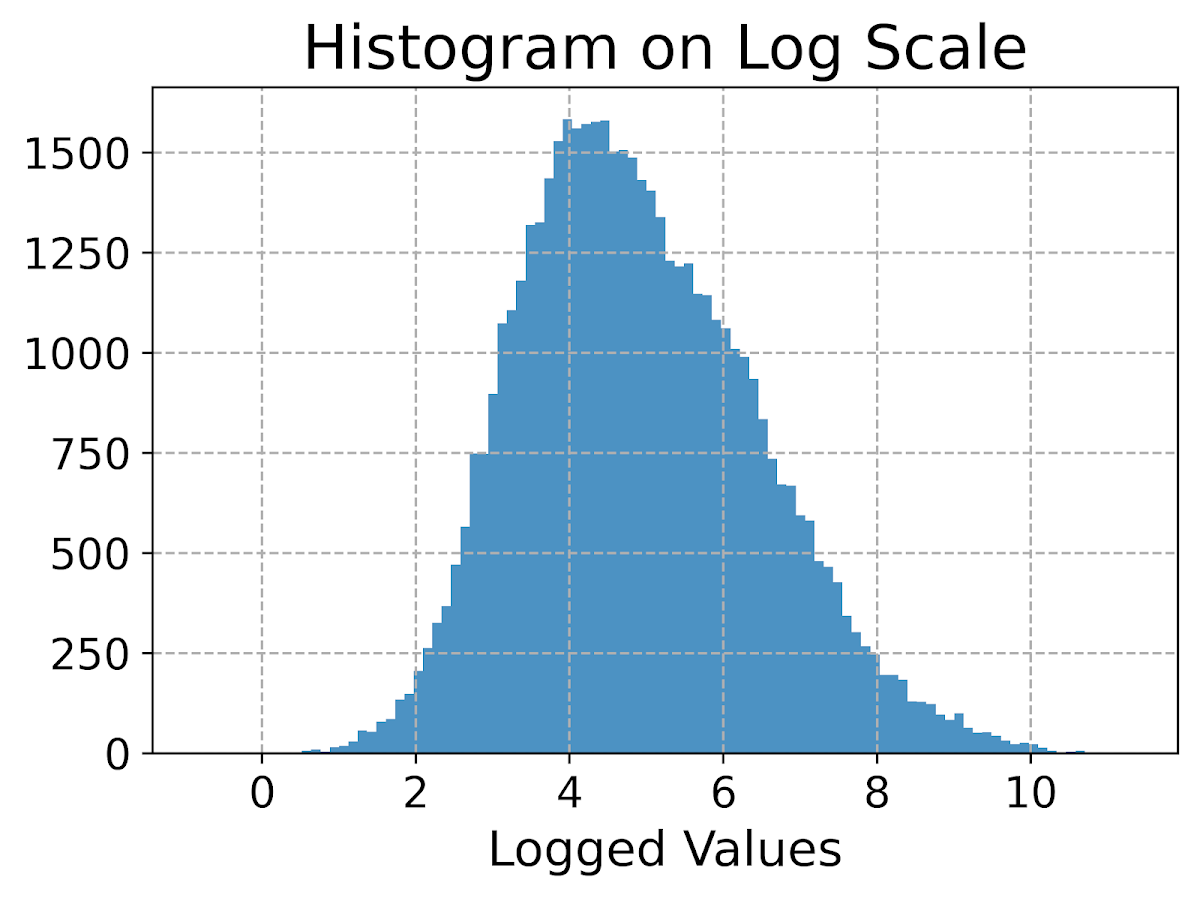Although it is hard to tell in this plot, the data are actually a mixture of three different log-normal distributions. One way to compare the distributions of different groups are by using groupby before the histogram call. #Using groupby to superimpose histograms dat.groupby('group')['log_vals'].hist(bins=100)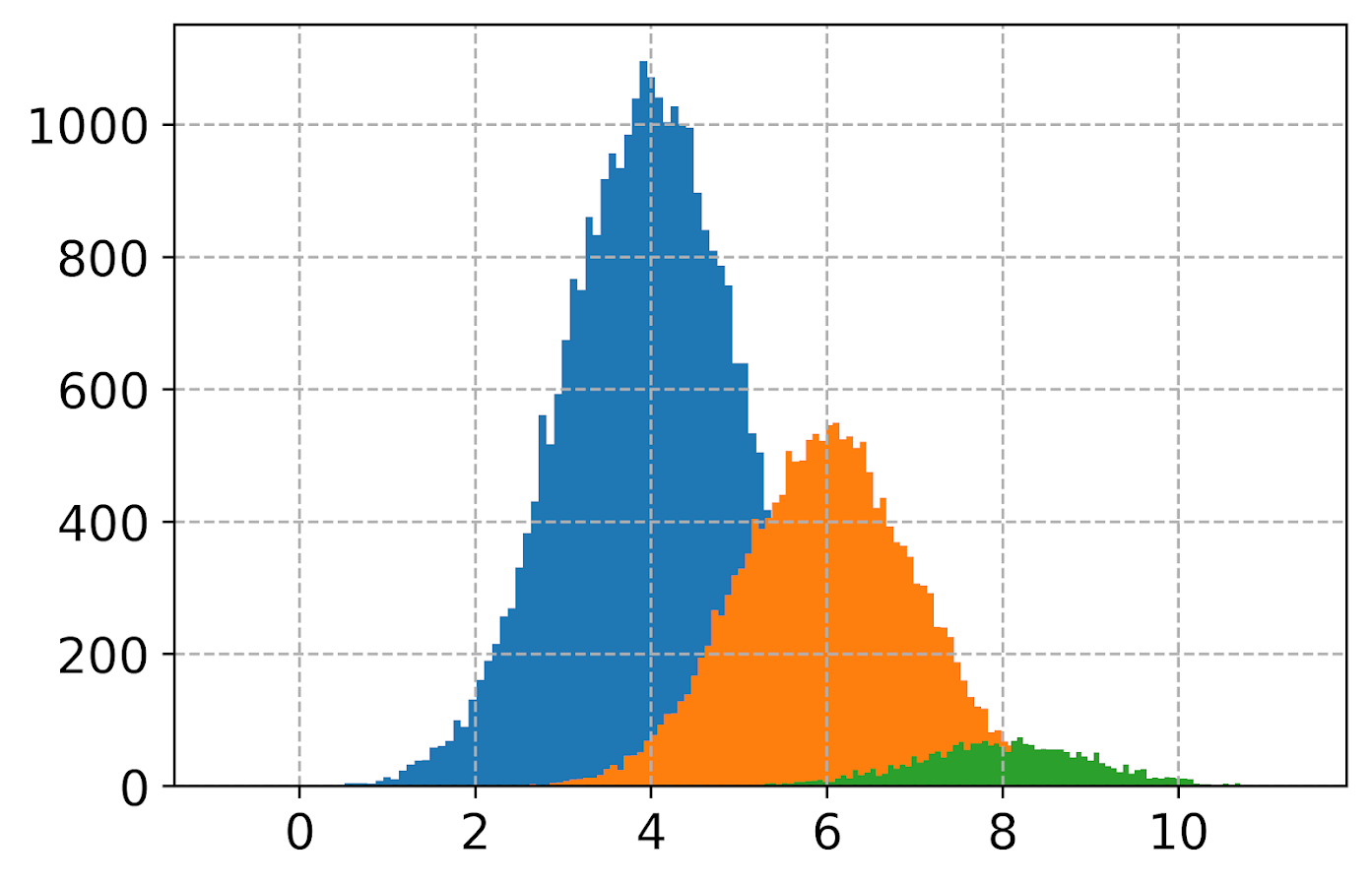But you see here two problems, since the groups are not near the same size, some are shrunk in the plot. The second is I don’t know which group is which. To normalize the areas for each subgroup, specifying the density option is one solution. Also plotting at a higher alpha level lets you see the overlaps a bit more clearly. dat.groupby('group')['log_vals'].hist(bins=100, alpha=0.65, density=True)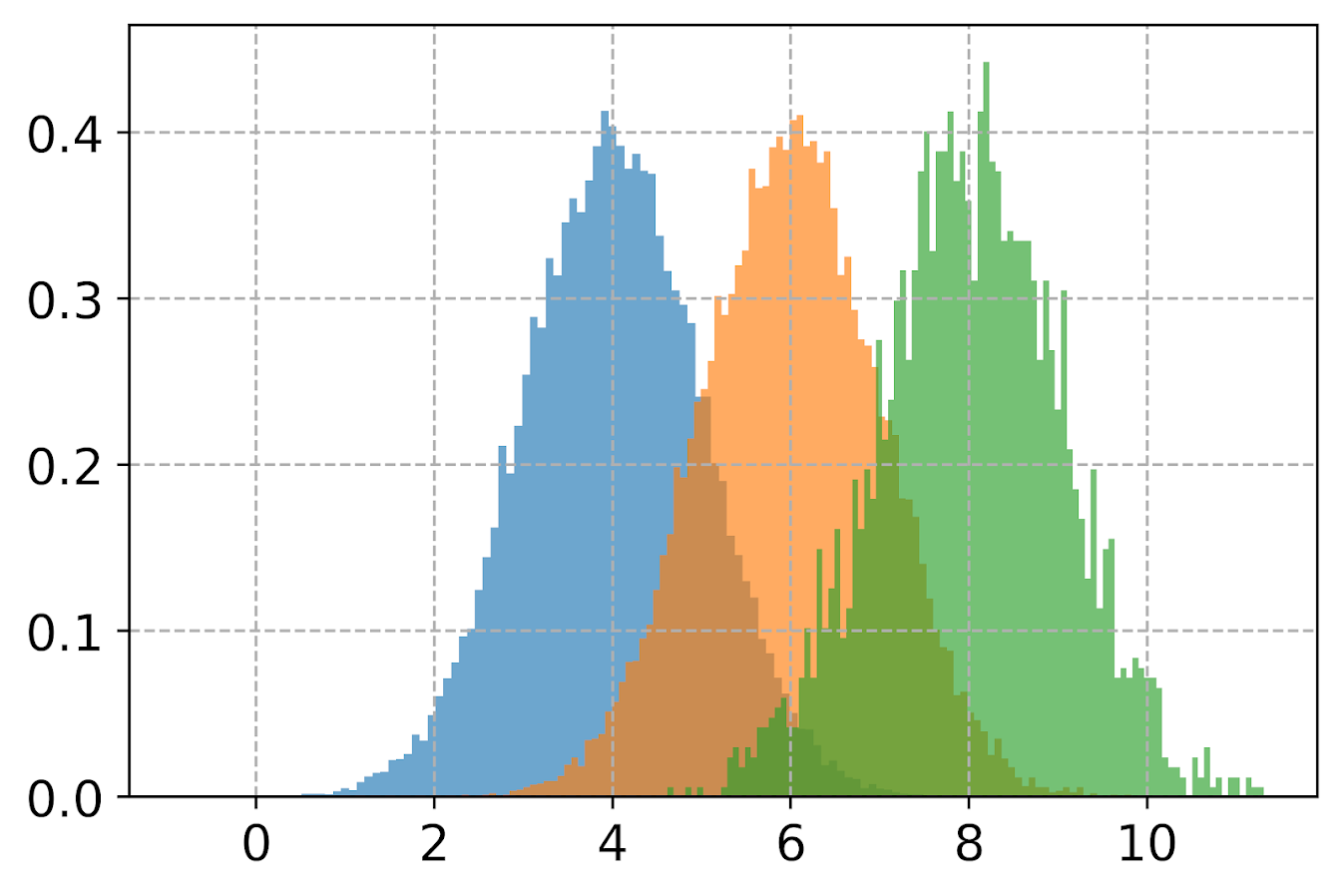Unfortunately I keep getting an error when I specify legend=True within the hist() function, and specifying plt.legend after the call just results in an empty legend. So another option is to do a small multiple plot, by specifying a by option within the hist function (instead of groupby). #Small multiple plot dat['log_vals'].hist(bins=100, by=dat['group'], alpha=0.8, figsize=(8,8))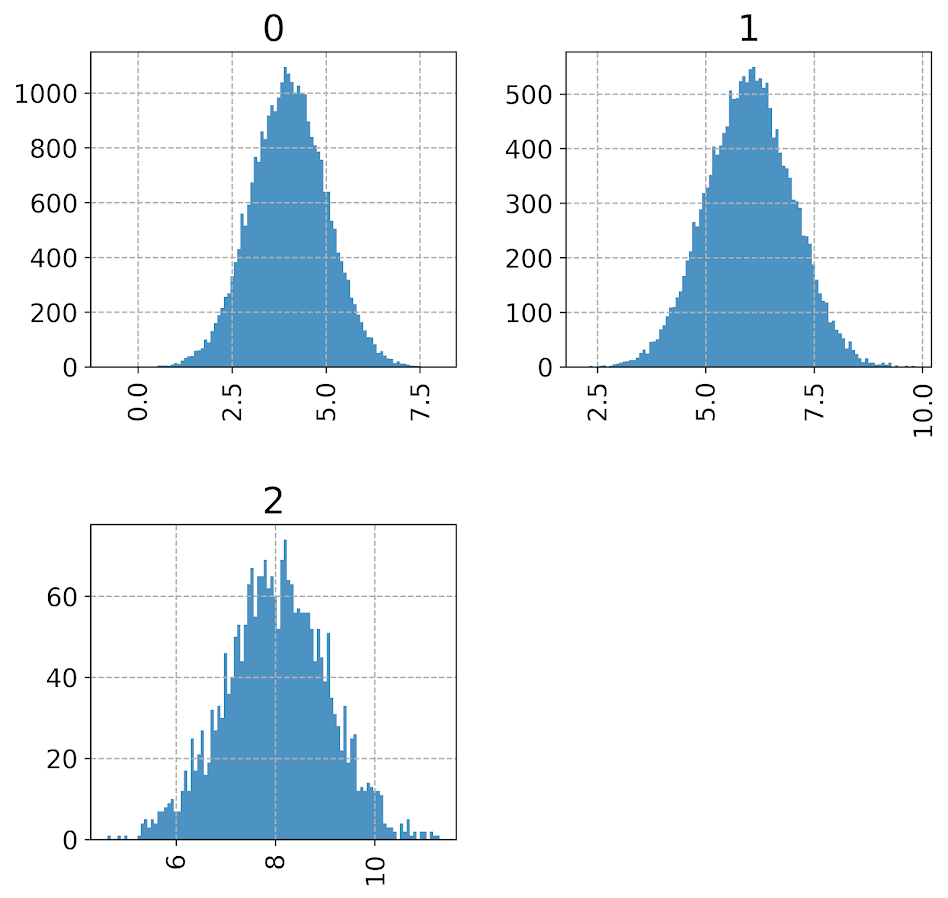This takes up more room, so can pass in the figsize() parameter directly to expand the area of the plot. Be careful when interpreting these, as all the axes are by default not shared, so both the Y and X axes are different, making it harder to compare offhand. Going back to the superimposed histograms, to get the legend to work correctly this is the best solution I have come up with, just simply creating different charts in a loop based on the subset of data. (I think that is easier than building the legend yourself.) #Getting the legend to work! for g in pd.unique(dat['group']): dat.loc[dat['group']==g,'log_vals'].hist(bins=100,alpha=0.65, label=g,density=True) plt.legend(loc='upper left')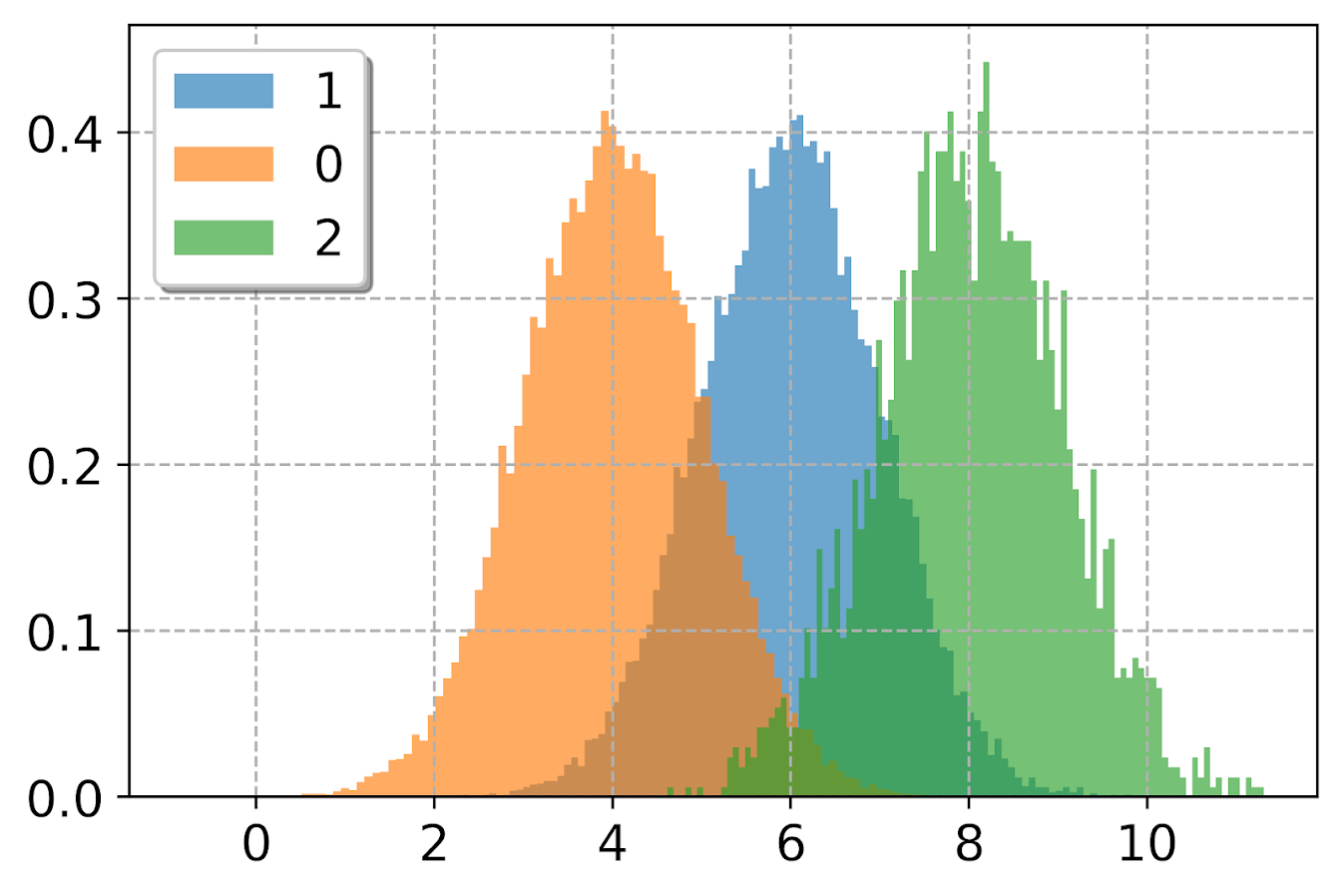Besides the density=True to get the areas to be the same size, another trick that can sometimes be helpful is to weight the statistics by the inverse of the group size. The Y axis is not really meaningful here, but this sometimes is useful for other chart stats as well. #another trick, inverse weighting dat['inv_weights'] = 1/dat.groupby('group')['vals'].transform('count') for g in pd.unique(dat['group']): sub_dat = dat[dat['group']==g] sub_dat['log_vals'].hist(bins=100,alpha=0.65, label=g,weights=sub_dat['inv_weights']) plt.legend(loc='upper left')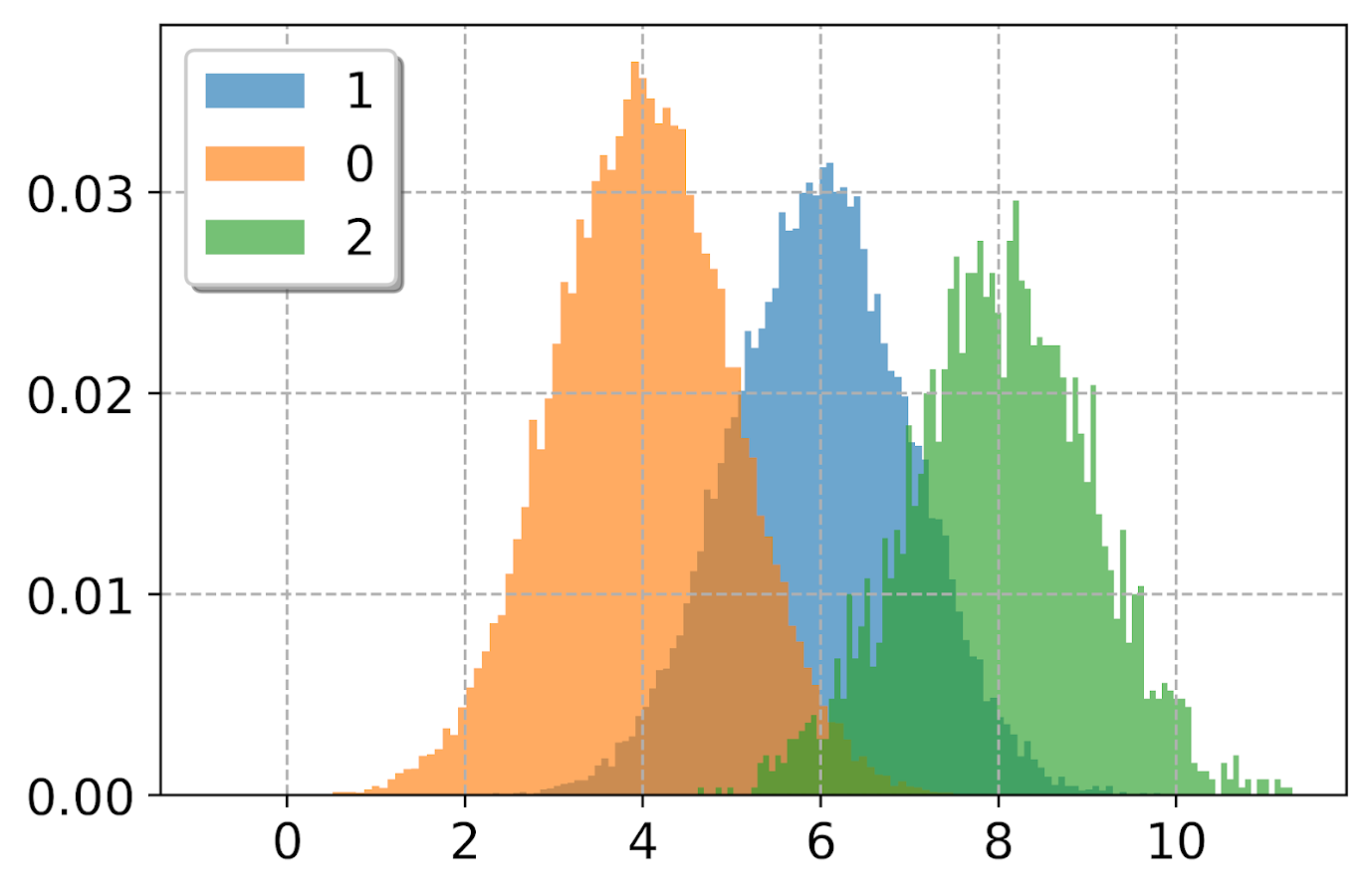So far, I have plotted the logged values. But I often want the labels to show the original values, not the logged ones. There are two different ways to deal with that. One is to plot the original values, but then use a log scale axis. When you do it this way, you want to specify your own bins for the histogram. Here I also show how you can use StrMethodFormatter to return a money value. Also rotate the labels so they do not collide. #Specifying your own bins on original scale #And using log formatting log_bins = np.exp(np.arange(0,12.1,0.1)) ax = dat['vals'].hist(bins=log_bins, alpha=0.8) plt.xscale('log', basex=10) ax.xaxis.set_major_formatter(StrMethodFormatter('${x:,.0f}'))
plt.xticks(rotation=45)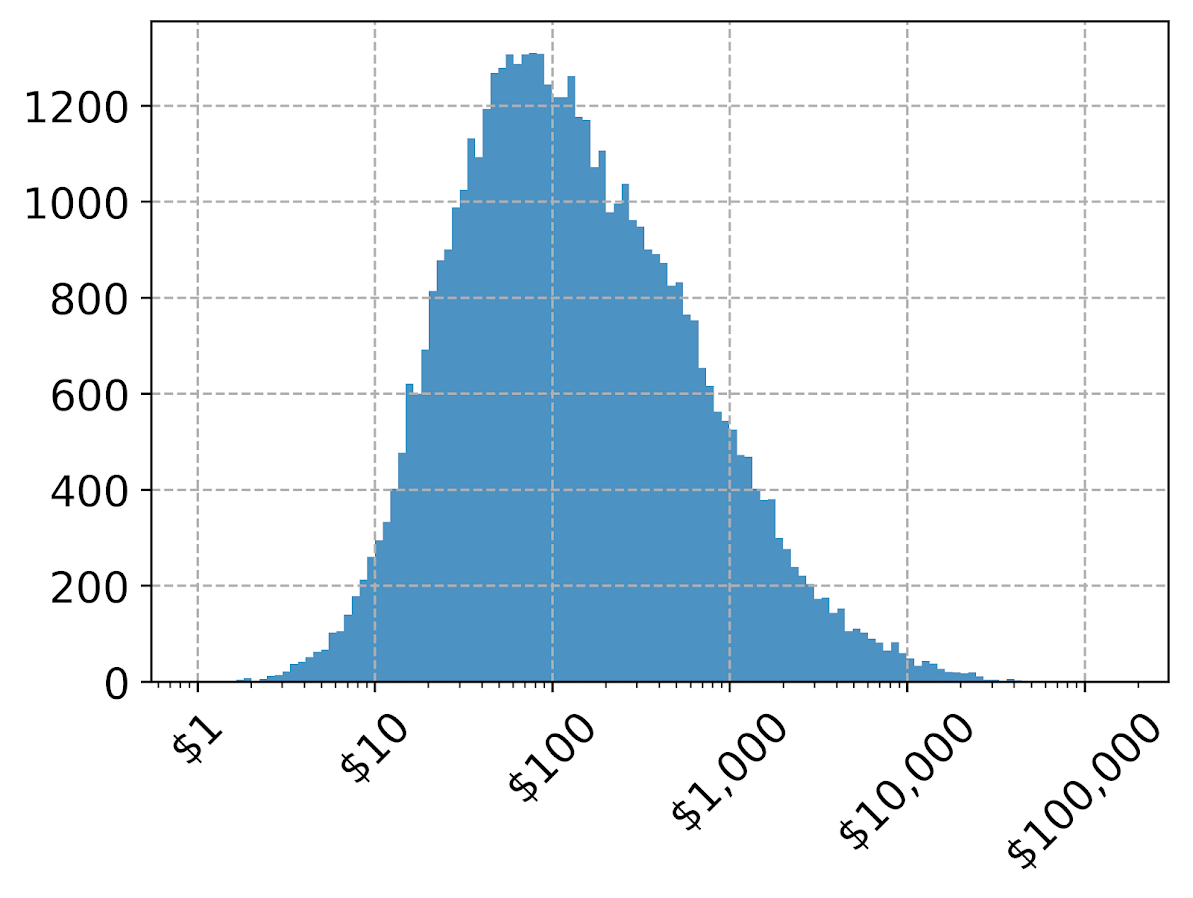If you omit the formatter option, you can see the returned values are 10^2, 10^3 etc. Besides log base 10, folks should often give log base 2 or log base 5 a shot for your data.

Another way though is to use our original logged values, and change the format in the chart. Here we can do that using FuncFormatter.

#Using the logged scaled, then a formatter
#https://napsterinblue.github.io/notes/python/viz/tick_string_formatting/
def exp_fmt(x,pos):
return '\${:,.0f}'.format(np.exp(x))
fmtr = FuncFormatter(exp_fmt)

ax = dat['log_vals'].hist(bins=100, alpha=0.8)
plt.xticks(np.log([5**i for i in range(7)]))
ax.xaxis.set_major_formatter(fmtr)
plt.xticks(rotation=45)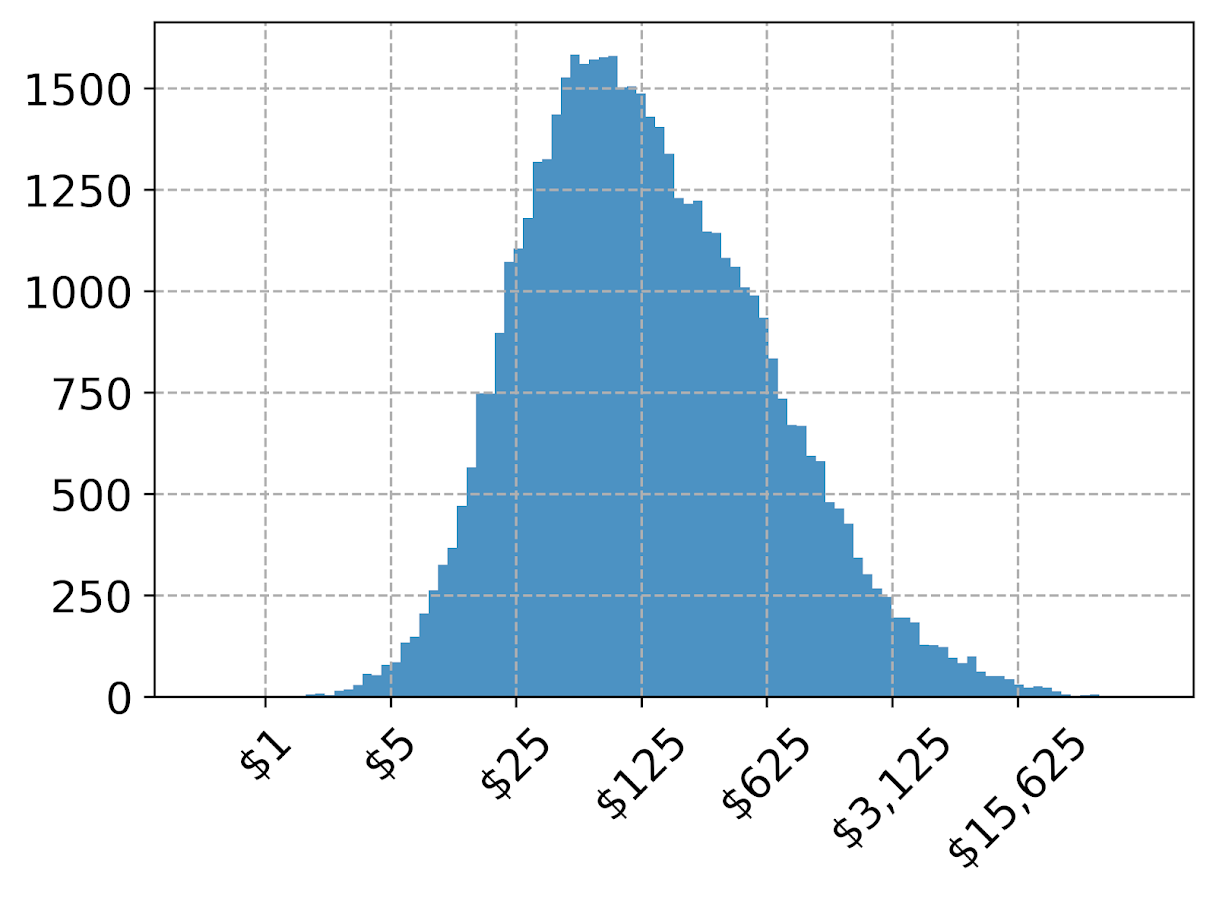On the slate is to do some other helpers for scatterplots and boxplots. The panda defaults are no doubt good for EDA, but need some TLC to make more presentation ready.